## Background

We performed a series of tests on English Wikipedia requesting feedback from users about whether the article they were reading was relevant to one of the curated search queries. For our minimum viable product (MVP) test, we hard-coded a list of queries and articles. We also tried different wordings of the relevance question, to assess the impact of each.Uploaded to Phabricator by Erik Bernhardson (F9161493)

For this MVP, the queries were chosen to be about topics for which we could confidently judge an article’s relevance beforehand, such as American pop culture:

• who is v for vendetta?
• star and stripes
• block buster
• 10 items or fewer
• sailor soldier tinker spy
• how do flowers bloom?
• yesterday beetles
• search engine
• what is a genius iq?
• why is a baby goat a kid?

For each query, we judged the relevance of the articles that were the top 5 results for the queries at the time (and most are still the top 5 results). The following table shows which pages we asked users about and our judgements:

query article opinion
who is v for vendetta? V for Vendetta (film) ok
V for Vendetta ok
List of V for Vendetta characters good
V (comics) best
star and stripes Stars and Stripes Forever (disambiguation) ok
The Stars and Stripes Forever ok
block buster Blockbuster best
Block Buster! good
Block Busters ok
10 items or fewer Fewer vs. less ok
Magic item (Dungeons & Dragons) very bad
sailor soldier tinker spy Tinker Tailor Soldier Spy best
Tinker, Tailor good
Blanket of Secrecy ok
List of fictional double agents ok
Ian Bannen ok
how do flowers bloom? Britain in Bloom bad
Flowers in the Attic (1987 film) very bad
Flower best
Flowers in the Attic very bad
yesterday beetles Private language argument very bad
How Do You Sleep? (John Lennon song) very bad
The Collected Stories of Philip K. Dick very bad
search engine Web search engine best
List of search engines good
Search engine optimization ok
Search engine marketing ok
Audio search engine ok
what is a genius iq? Genius good
IQ classification best
High IQ society ok
Social IQ score of bacteria bad
why is a baby goat a kid? Goat best
The Kids from Room 402 very bad

A user visiting one of those articles might be randomly picked for the survey. There were 4 varieties of questions that we asked:

2. If you searched for ‘…’, would this article be a good result?

(Where … was replaced with the actual query.)

The variations on the questions were so we could assess how the wording/phrasing affected the results.

## Results

### First Test

aggregates_first <- responses_first %>%
dplyr::group_by(query, article, question, choice) %>%
dplyr::tally() %>%
dplyr::ungroup() %>%
tidyr::spread(choice, n, fill = 0) %>%
dplyr::mutate(
total = yes + no,
score = (yes - no) / (total + 1),
yes = yes / total,
no = no / total,
dismiss = dismiss / (total + dismiss),
engaged = (total + dismiss) / (total + dismiss + timeout)
) %>%
dplyr::select(-c(total, timeout)) %>%
tidyr::gather(choice, prop, -c(query, article, question)) %>%
dplyr::mutate(choice = factor(choice, levels = c("yes", "no", "dismiss", "engaged", "score")))

#### Summary

The first test (08/04-08/10) had 0 time delay and presented users with options to answer “Yes”, “No”, “I don’t know”, or dismiss the notification. The notification disappeared after 30 seconds if the user did not interact with it. Due to a bug, the “I don’t know” responses were not recorded for this test. There were 11,056 sessions and 3,016 yes/no responses. 8,703 (73.6%) surveys timed out and 100 surveys were dismissed by the user.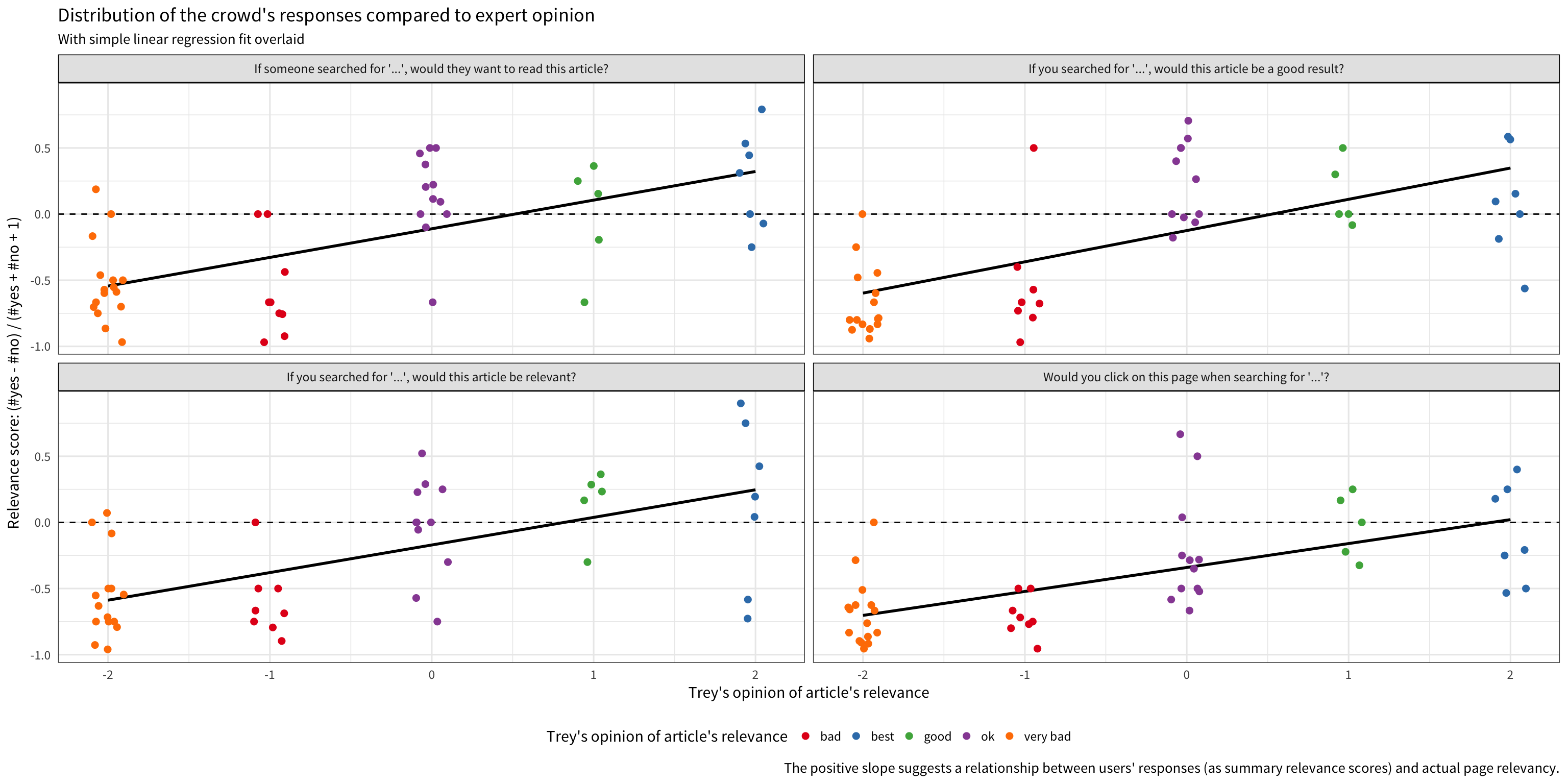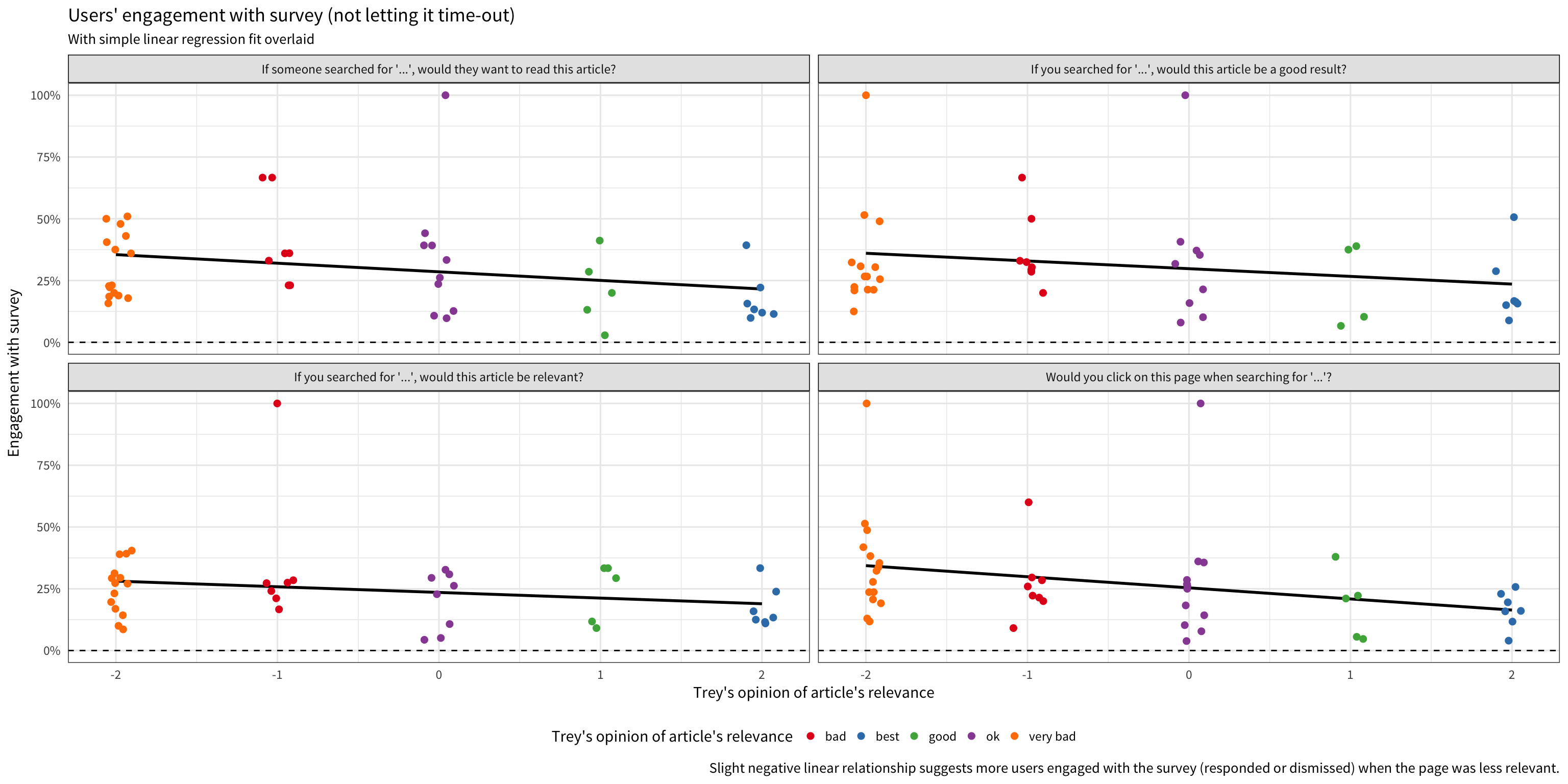↑ Top of section

#### Survey Responses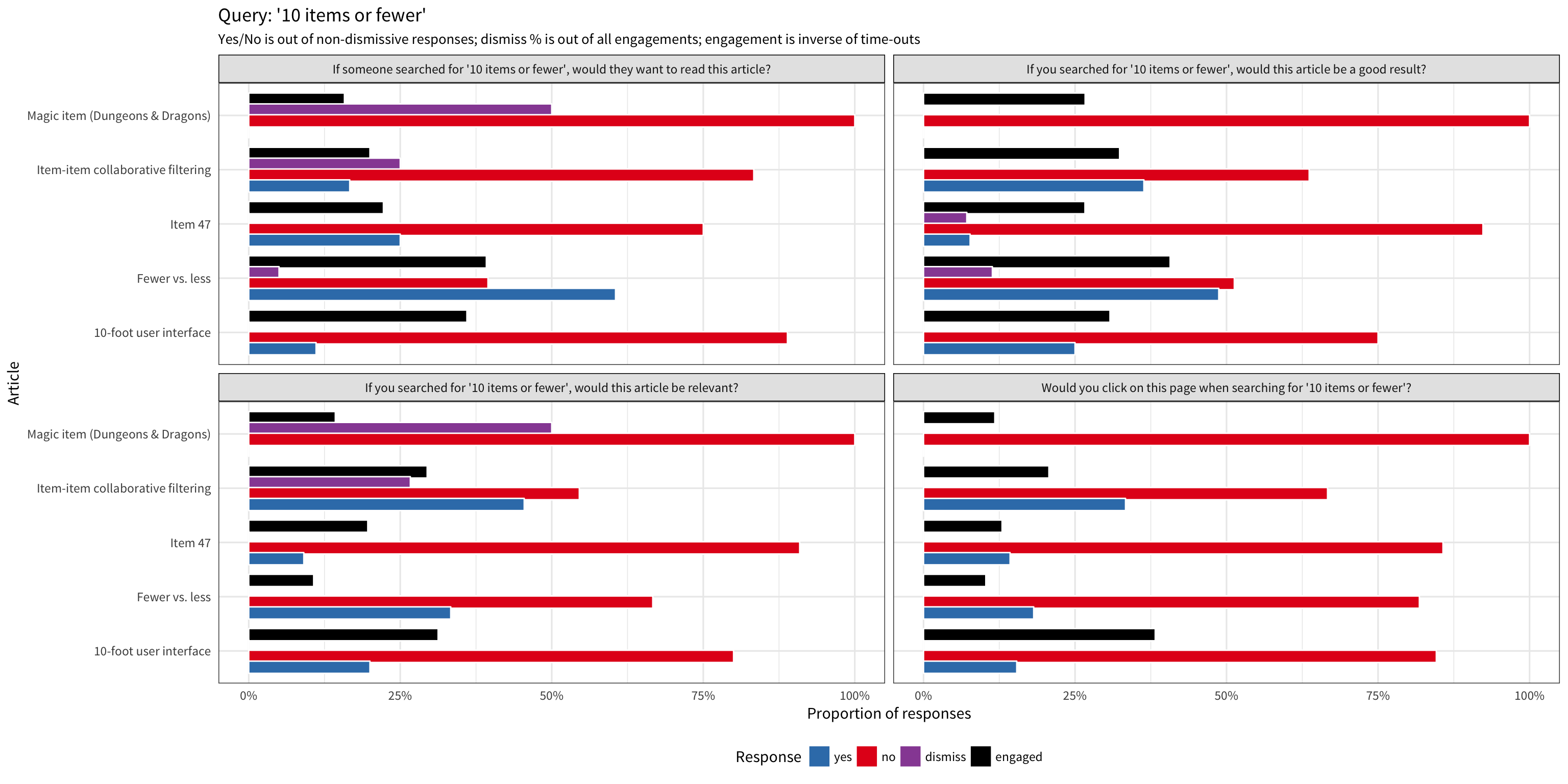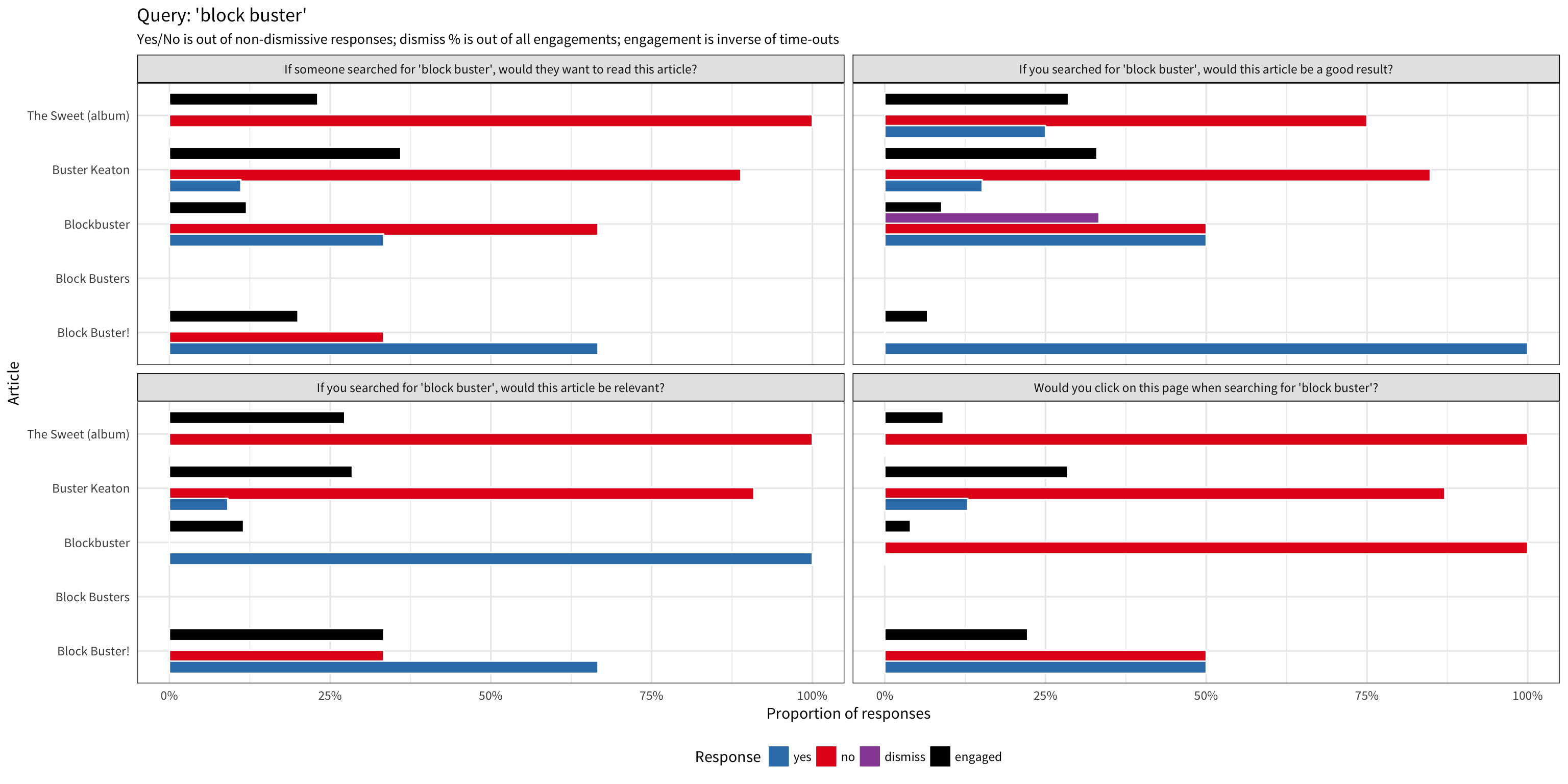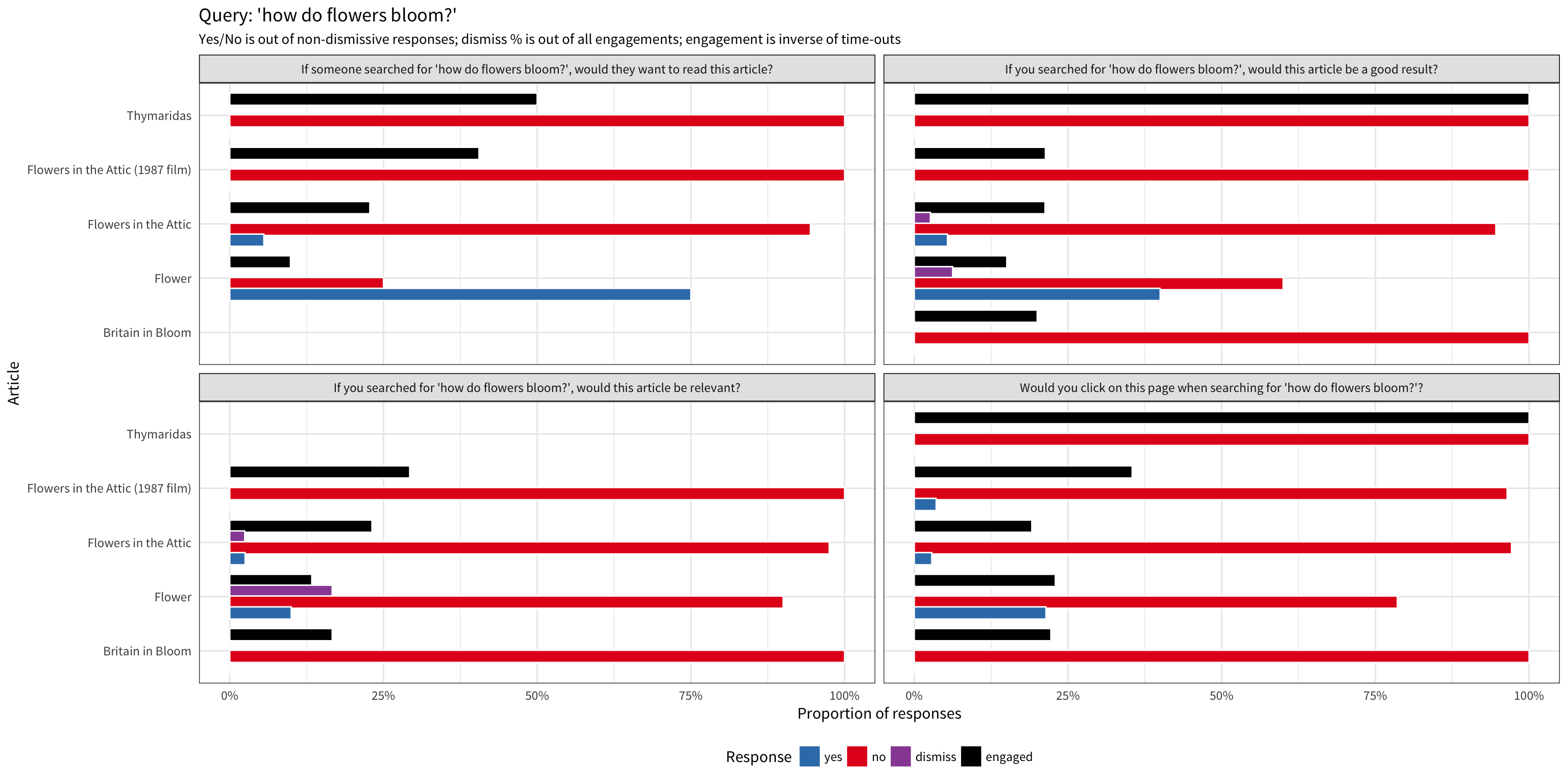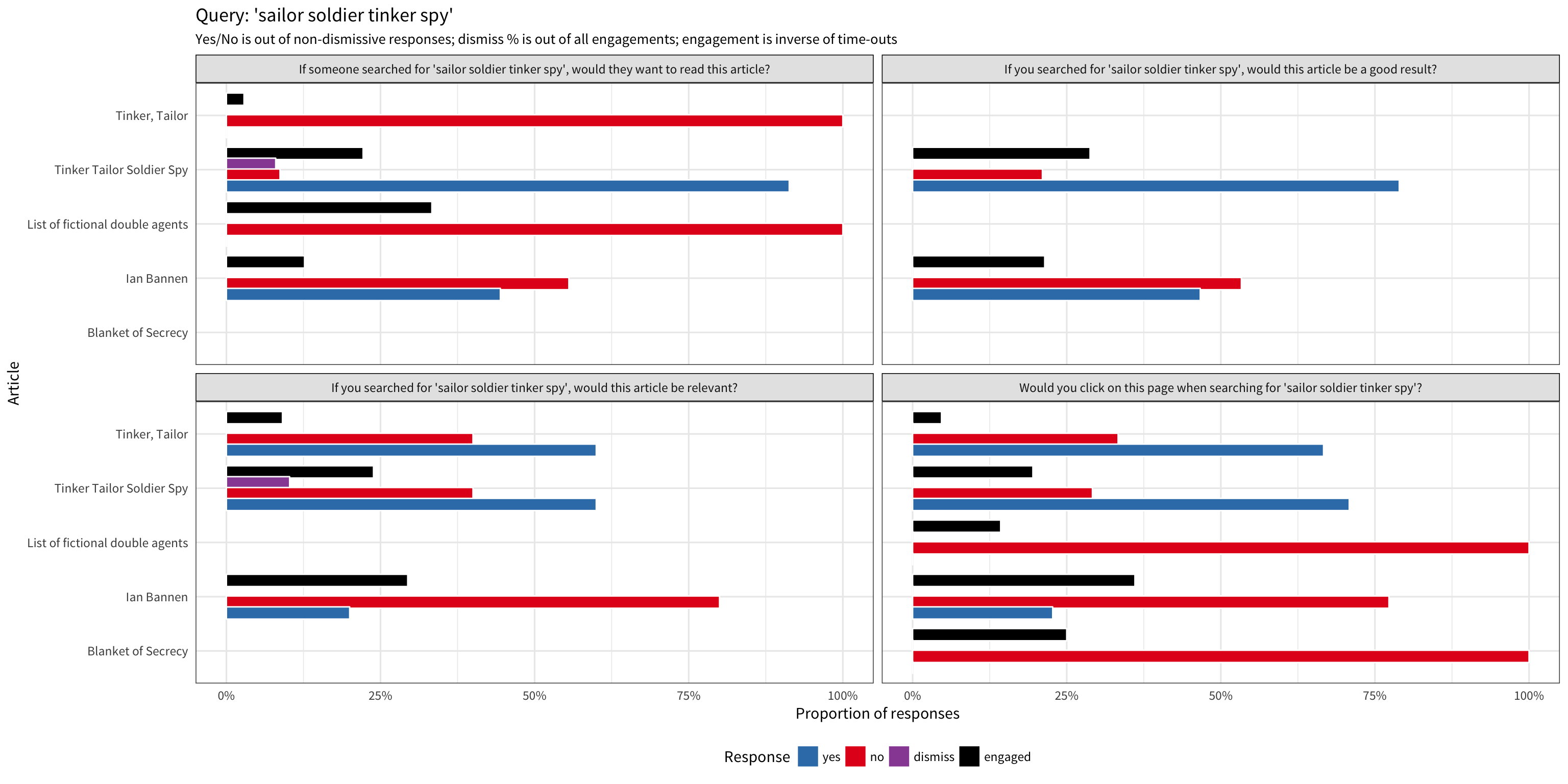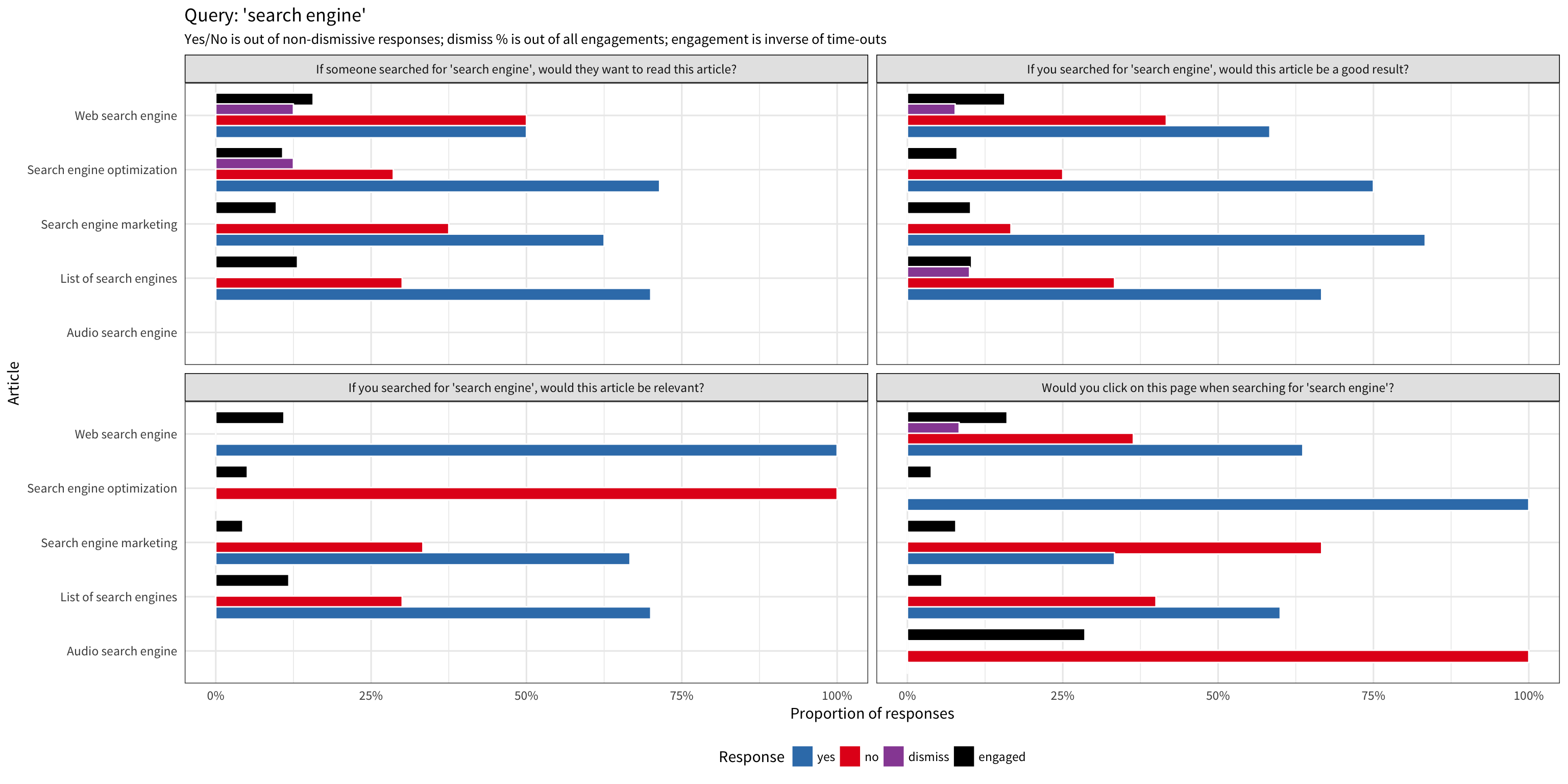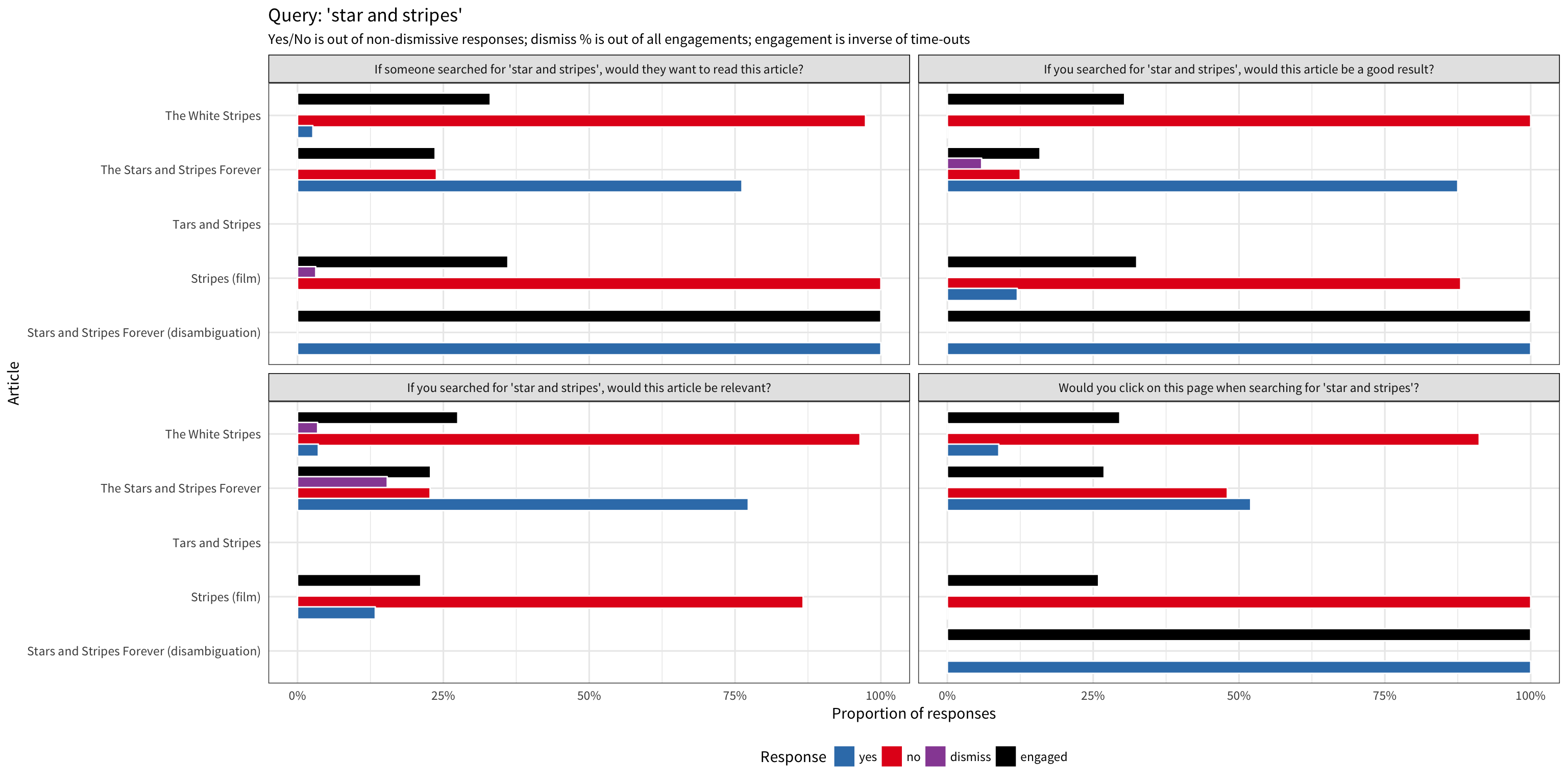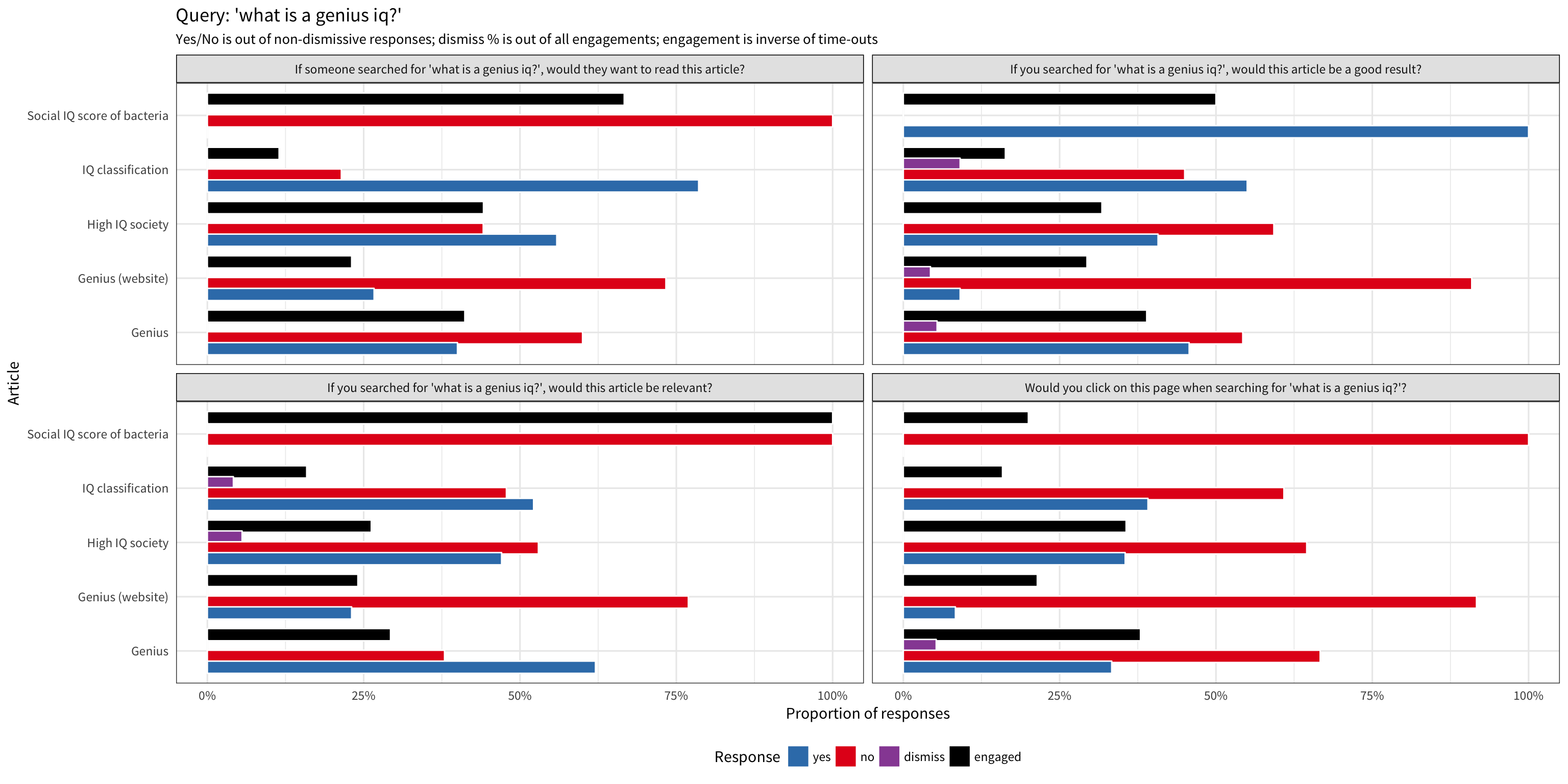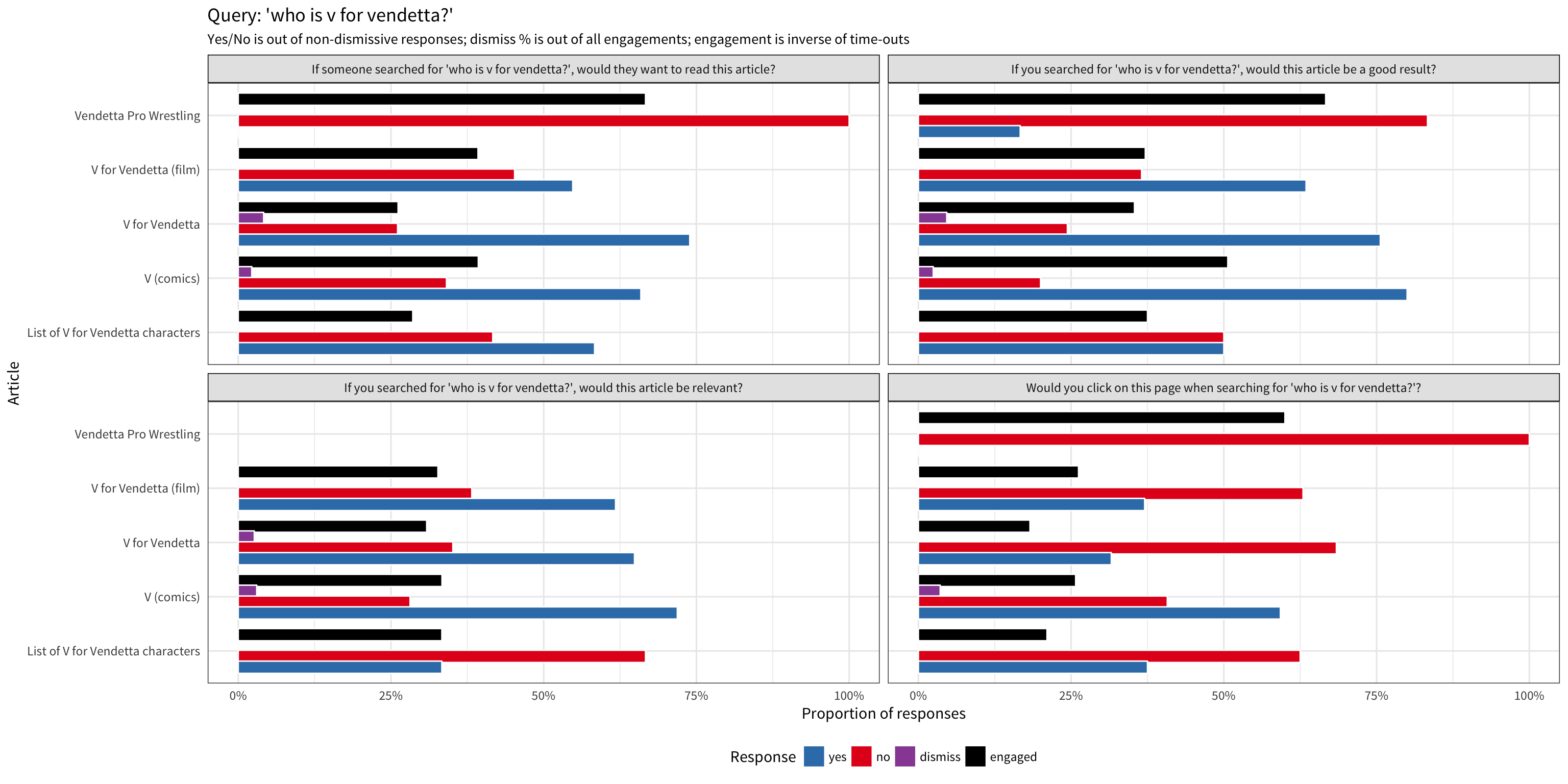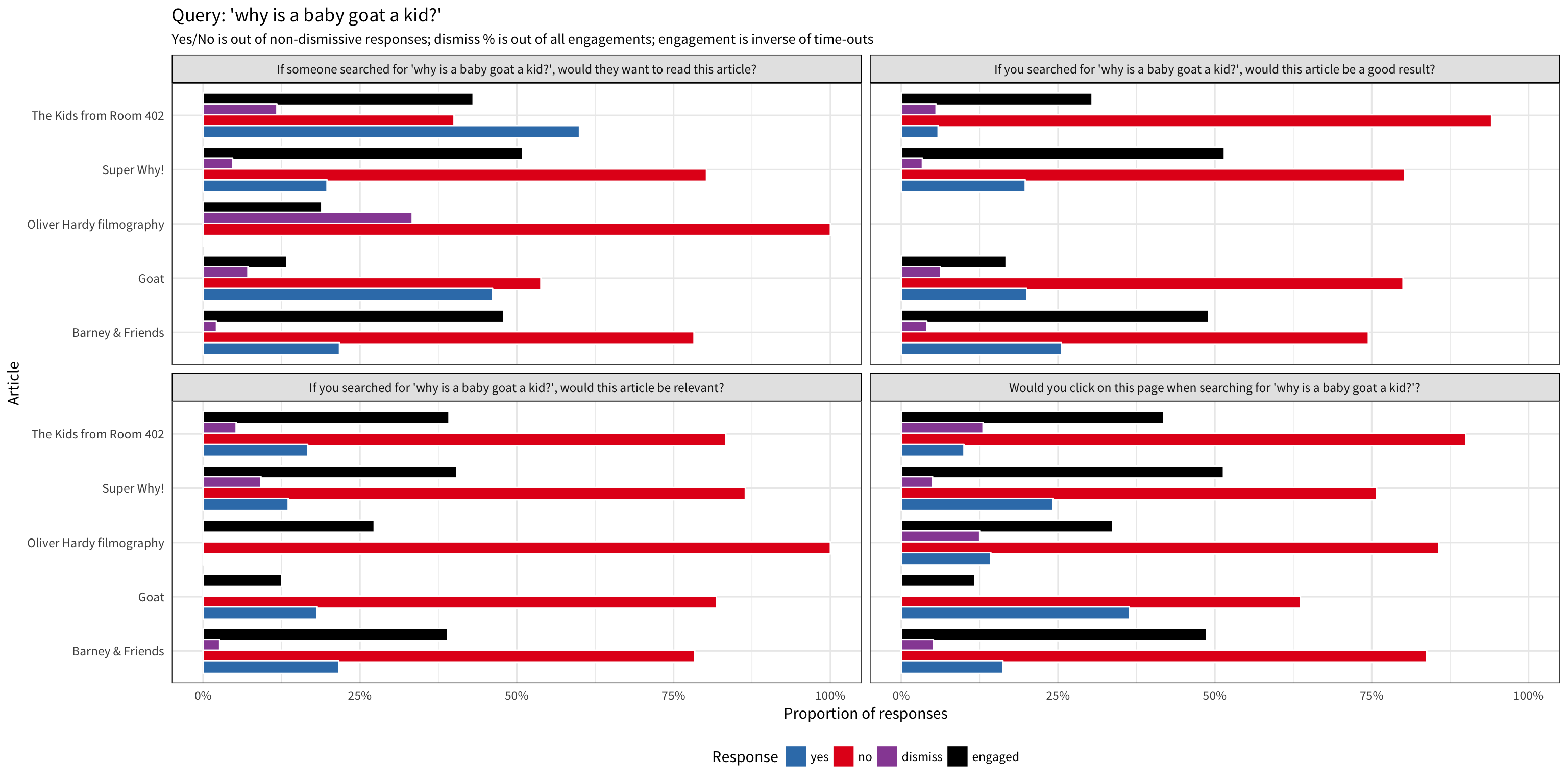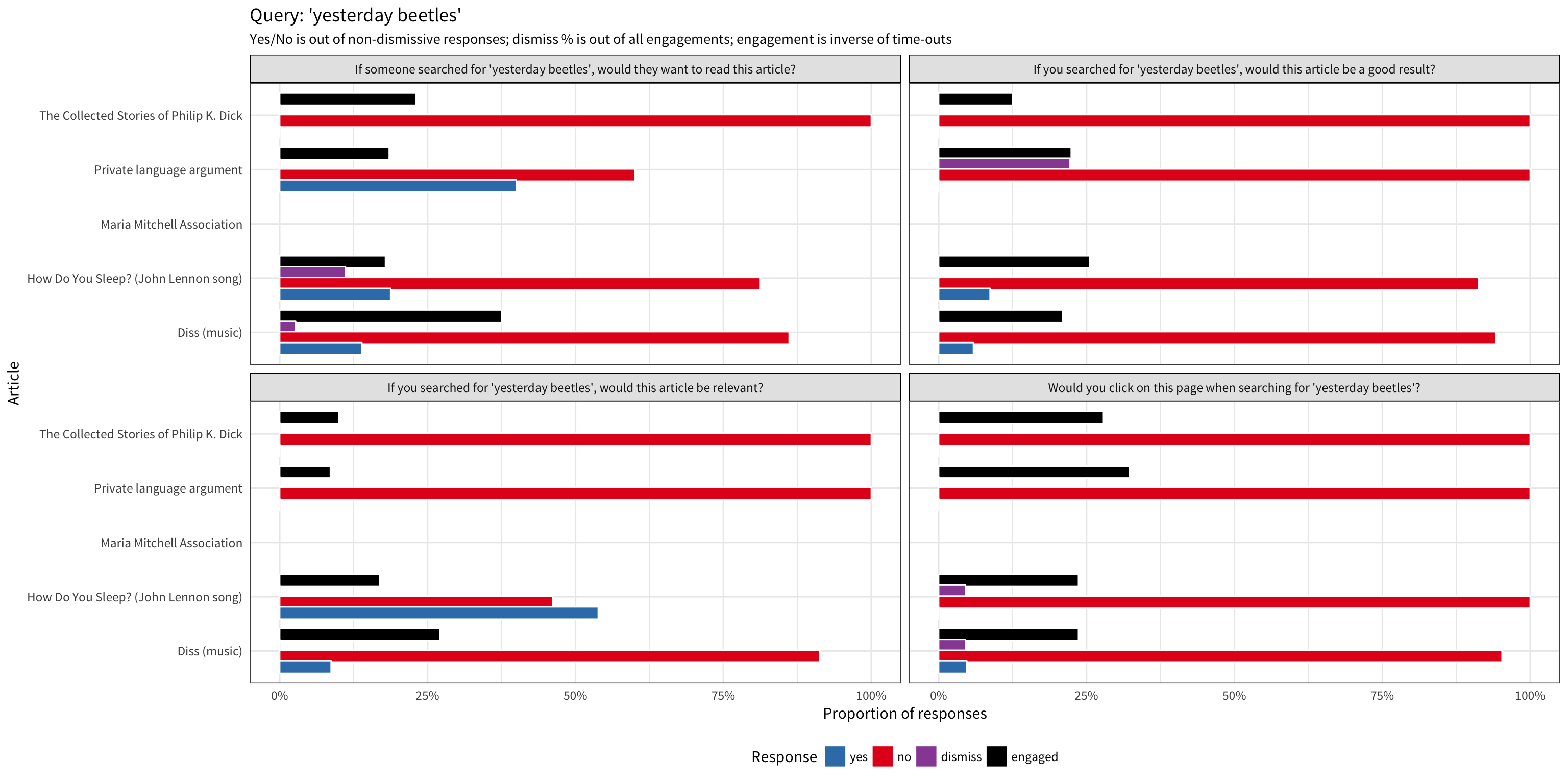↑ Top of section

#### Relevance Predictions

We want to be able to categorize articles as relevant/irrelevant based on user’s survey responses. We train a number of classification models using expert opinion as the response and a summary score and engagement as predictors. They are computed as follows:

$\text{Score} = \frac{\#\{\text{response: yes}\} - \#\{\text{response: no}\}}{\#\{\text{response: yes}\} + \#\{\text{response: no}\} + 1}$

$\text{Engagement} = \frac{\#\{\text{response: yes/no/dismiss}\}}{\#\{\text{surveys}\}}$

The classifiers trained are:

We trained a classifier on each of the 4 questions using the default parameters and a random 70% of the pages as training data and assess its accuracy using a test set from the remaining 30% of the pages. We tried 5-class, 3-class, and 2-class models. The 5-label classification performed the worst due to not enough data per-class, which is why there is a substantial improvement when we grouped combined “very bad” with “bad” and combined “good” with “best” to create the 3 classes. Best performances were with binary (irrelevant = very bad / bad, relevant = ok / good / best) classification. Classifiers trained on responses to questions 1 and 4 had the highest accuracy.

set.seed(42)
ratings_first <- responses_first %>%
dplyr::mutate(
question = as.numeric(factor(question, levels = c(
"If you searched for '...', would this article be a good result?",
)))
) %>%
dplyr::group_by(query, article, choice, question) %>%
dplyr::tally() %>%
dplyr::ungroup() %>%
tidyr::spread(choice, n, fill = 0) %>%
dplyr::mutate(
total = yes + no + 1,
engaged = yes + no + dismiss,
score = (yes - no) / total,
engagement = (engaged + dismiss) / (total + dismiss + timeout),
# Normalized versions:
score_norm = (score - mean(score)) / sd(score),
engagement_norm = (engagement - mean(engagement)) / sd(engagement)
) %>%
dplyr::left_join(trey, by = c("query", "article")) %>%
dplyr::mutate(
ok_or_better = as.numeric(opinion %in% c("ok", "good", "best")),
relevant = as.numeric(opinion %in% c("good", "best")),
ok = as.numeric(opinion == "ok"),
good = as.numeric(opinion == "good"),
best = as.numeric(opinion == "best"),
opinion2 = factor(dplyr::case_when(
opinion %in% c("ok", "good", "best") ~ "ok or better"
), levels = c("bad", "ok or better")),
opinion3 = factor(dplyr::case_when(
opinion %in% c("good", "best") ~ "good",
opinion == "ok" ~ "ok"
), levels = c("bad", "ok", "good")),
) %>%
split(., .$question) %>% lapply(function(df) { training_idx <- sample.int(nrow(df), 0.7 * nrow(df), replace = FALSE) testing_idx <- setdiff(1:nrow(df), training_idx) return(list(train = df[training_idx, ], test = df[testing_idx, ])) }) set.seed(0) logistic_regression <- dplyr::bind_rows(list( "2" = dplyr::bind_rows(lapply(ratings_first, function(question) { lr <- glm(opinion2 ~ score + engagement, data = question$train, family = binomial())
predictions <- predict(lr, question$test[, c("score", "engagement")], type = "response") return(data.frame(accuracy = caret::confusionMatrix( factor(predictions > 0.5, c(FALSE, TRUE), levels(question$test$opinion2)), reference = question$test$opinion2 )$overall["Accuracy"]))
}), .id = "question"),
"3" = dplyr::bind_rows(lapply(ratings_first, function(question) {
lr <- nnet::multinom(
opinion3 ~ score + engagement,
data = question$train, trace = FALSE ) predictions <- predict(lr, question$test[, c("score", "engagement")])
return(data.frame(accuracy = caret::confusionMatrix(
predictions, reference = question$test$opinion3
)$overall["Accuracy"])) }), .id = "question"), "5" = dplyr::bind_rows(lapply(ratings_first, function(question) { lr <- nnet::multinom( opinion5 ~ score + engagement, data = question$train,
trace = FALSE
)
predictions <- predict(lr, question$test[, c("score", "engagement")]) return(data.frame(accuracy = caret::confusionMatrix( predictions, reference = question$test$opinion5 )$overall["Accuracy"]))
}), .id = "question")
), .id = "categories")

random_forest <- dplyr::bind_rows(list(
"2" = dplyr::bind_rows(lapply(ratings_first, function(question) {
rf <- randomForest::randomForest(
opinion2 ~ score + engagement,
data = question$train, ntree = 1000 ) predictions <- predict(rf, question$test[, c("score", "engagement")])
return(data.frame(accuracy = caret::confusionMatrix(
predictions, reference = question$test$opinion2
)$overall["Accuracy"])) }), .id = "question"), "3" = dplyr::bind_rows(lapply(ratings_first, function(question) { rf <- randomForest::randomForest( opinion3 ~ score + engagement, data = question$train,
ntree = 1000
)
predictions <- predict(rf, question$test[, c("score", "engagement")]) return(data.frame(accuracy = caret::confusionMatrix( predictions, reference = question$test$opinion3 )$overall["Accuracy"]))
}), .id = "question"),
"5" = dplyr::bind_rows(lapply(ratings_first, function(question) {
rf <- randomForest::randomForest(
opinion5 ~ score + engagement,
data = question$train, ntree = 1000 ) predictions <- predict(rf, question$test[, c("score", "engagement")])
return(data.frame(accuracy = caret::confusionMatrix(
predictions, reference = question$test$opinion5
)$overall["Accuracy"])) }), .id = "question") ), .id = "categories") neural_net <- dplyr::bind_rows(list( "2" = dplyr::bind_rows(lapply(ratings_first, function(question) { nn <- neuralnet::neuralnet( irrelevant + ok_or_better ~ score + engagement, data = question$train,
hidden = c(5, 3), stepmax = 1e6
)
predictions <- factor(
c("bad", "ok or better")[apply(neuralnet::compute(nn, question$test[, c("score", "engagement")])$net.result, 1, which.max)],
levels = c("bad", "ok or better")
)
return(data.frame(accuracy = caret::confusionMatrix(
predictions, reference = question$test$opinion2
)$overall["Accuracy"])) }), .id = "question"), "3" = dplyr::bind_rows(lapply(ratings_first, function(question) { nn <- neuralnet::neuralnet( irrelevant + ok + relevant ~ score + engagement, data = question$train,
hidden = c(5, 3), stepmax = 1e6
)
predictions <- factor(
c("bad", "ok", "good")[apply(neuralnet::compute(nn, question$test[, c("score", "engagement")])$net.result, 1, which.max)],
)
return(data.frame(accuracy = caret::confusionMatrix(
predictions, reference = question$test$opinion3
)$overall["Accuracy"])) }), .id = "question"), "5" = dplyr::bind_rows(lapply(ratings_first, function(question) { nn <- neuralnet::neuralnet( very_bad + bad + ok + good + best ~ score + engagement, data = question$train,
hidden = c(5, 3), stepmax = 1e6
)
predictions <- factor(
c("very bad", "bad", "ok", "good", "best")[apply(neuralnet::compute(nn, question$test[, c("score", "engagement")])$net.result, 1, which.max)],
)
return(data.frame(accuracy = caret::confusionMatrix(
predictions, reference = question$test$opinion5
)$overall["Accuracy"])) }), .id = "question") ), .id = "categories") naive_bayes <- dplyr::bind_rows(list( "2" = dplyr::bind_rows(lapply(ratings_first, function(question) { nb <- e1071::naiveBayes( opinion2 ~ score + engagement, data = question$train
)
predictions <- predict(nb, question$test[, c("score", "engagement")]) return(data.frame(accuracy = caret::confusionMatrix( predictions, reference = question$test$opinion2 )$overall["Accuracy"]))
}), .id = "question"),
"3" = dplyr::bind_rows(lapply(ratings_first, function(question) {
nb <- e1071::naiveBayes(
opinion3 ~ score + engagement,
data = question$train ) predictions <- predict(nb, question$test[, c("score", "engagement")])
return(data.frame(accuracy = caret::confusionMatrix(
predictions, reference = question$test$opinion3
)$overall["Accuracy"])) }), .id = "question"), "5" = dplyr::bind_rows(lapply(ratings_first, function(question) { nb <- e1071::naiveBayes( opinion5 ~ score + engagement, data = question$train
)
predictions <- predict(nb, question$test[, c("score", "engagement")]) return(data.frame(accuracy = caret::confusionMatrix( predictions, reference = question$test$opinion5 )$overall["Accuracy"]))
}), .id = "question")
), .id = "categories")

"2" = dplyr::bind_rows(lapply(ratings_first, function(question) {
xgb <- xgboost::xgboost(
data = as.matrix(question$train[, c("score", "engagement")]), label = as.numeric(question$train$opinion2) - 1, objective = "binary:logistic", nrounds = 100, nthread = 4, verbose = 0 ) predictions <- predict(xgb, as.matrix(question$test[, c("score", "engagement")]))
return(data.frame(accuracy = caret::confusionMatrix(
factor(predictions > 0.5, c(FALSE, TRUE), c("bad", "ok or better")),
reference = question$test$opinion2
)$overall["Accuracy"])) }), .id = "question"), "3" = dplyr::bind_rows(lapply(ratings_first, function(question) { xgb <- xgboost::xgboost( data = as.matrix(question$train[, c("score", "engagement")]),
label = as.numeric(question$train$opinion3) - 1, num_class = 3,
objective = "multi:softmax", nrounds = 100, nthread = 4, verbose = 0
)
predictions <- predict(xgb, as.matrix(question$test[, c("score", "engagement")])) return(data.frame(accuracy = caret::confusionMatrix( factor(predictions, 0:2, levels(question$test$opinion3)), reference = question$test$opinion3 )$overall["Accuracy"]))
}), .id = "question"),
"5" = dplyr::bind_rows(lapply(ratings_first, function(question) {
xgb <- xgboost::xgboost(
data = as.matrix(question$train[, c("score", "engagement")]), label = as.numeric(question$train$opinion5) - 1, num_class = 5, objective = "multi:softmax", nrounds = 100, nthread = 4, verbose = 0 ) predictions <- predict(xgb, as.matrix(question$test[, c("score", "engagement")]))
return(data.frame(accuracy = caret::confusionMatrix(
factor(predictions, 0:4, levels(question$test$opinion5)),
reference = question$test$opinion5
)$overall["Accuracy"])) }), .id = "question") ), .id = "categories") ↑ Top of section ### Second Test aggregates_second <- responses_second %>% dplyr::group_by(query, article, question, choice) %>% dplyr::tally() %>% dplyr::ungroup() %>% tidyr::spread(choice, n, fill = 0) %>% dplyr::mutate( total = yes + no + unsure, score_A = (yes - no) / (yes + no + 1), score_B = (yes - no + unsure / 2) / (total + 1), yes = yes / total, no = no / total, unsure = unsure / total, dismiss = dismiss / (total + dismiss), engaged = (total + dismiss) / (total + dismiss + timeout) ) %>% dplyr::select(-c(total, timeout)) %>% tidyr::gather(choice, prop, -c(query, article, question)) %>% dplyr::mutate(choice = factor(choice, levels = c("yes", "no", "unsure", "dismiss", "engaged", "score_A", "score_B"))) temp <- dplyr::left_join( tidyr::spread(aggregates_second, choice, prop), trey, by = c("query", "article") ) #### Summary The second test (08/11-08/18) had a 60 second time delay and presented users with options to answer “Yes”/“No”/“I don’t know” (coded as “unsure”) or dismiss the notification. The notification disappeared after 30 seconds if the user did not interact with it. There were 7,828 sessions and 1,893 yes/no/unsure responses. 6,191 (76.3%) surveys timed out and 26 surveys were dismissed by the user. With the first test, it was easier to develop a scoring method using just the number of “yes” and “no” responses. With the second test, we had to include the “I don’t know” (coded as “unsure”) responses. Because of this, we came up with two possible scoring systems: method A does not count “unsure” responses but does use them to normalize the score; method B counts half of “unsure” responses and uses all three possible responses to normalize the score. Classifiers trained on responses to questions 3 and 4 appeared to have the highest accuracy. $\text{Engagement} = \frac{\#\{\text{response: yes/no/unsure/dismiss}\}}{\#\{\text{surveys}\}}$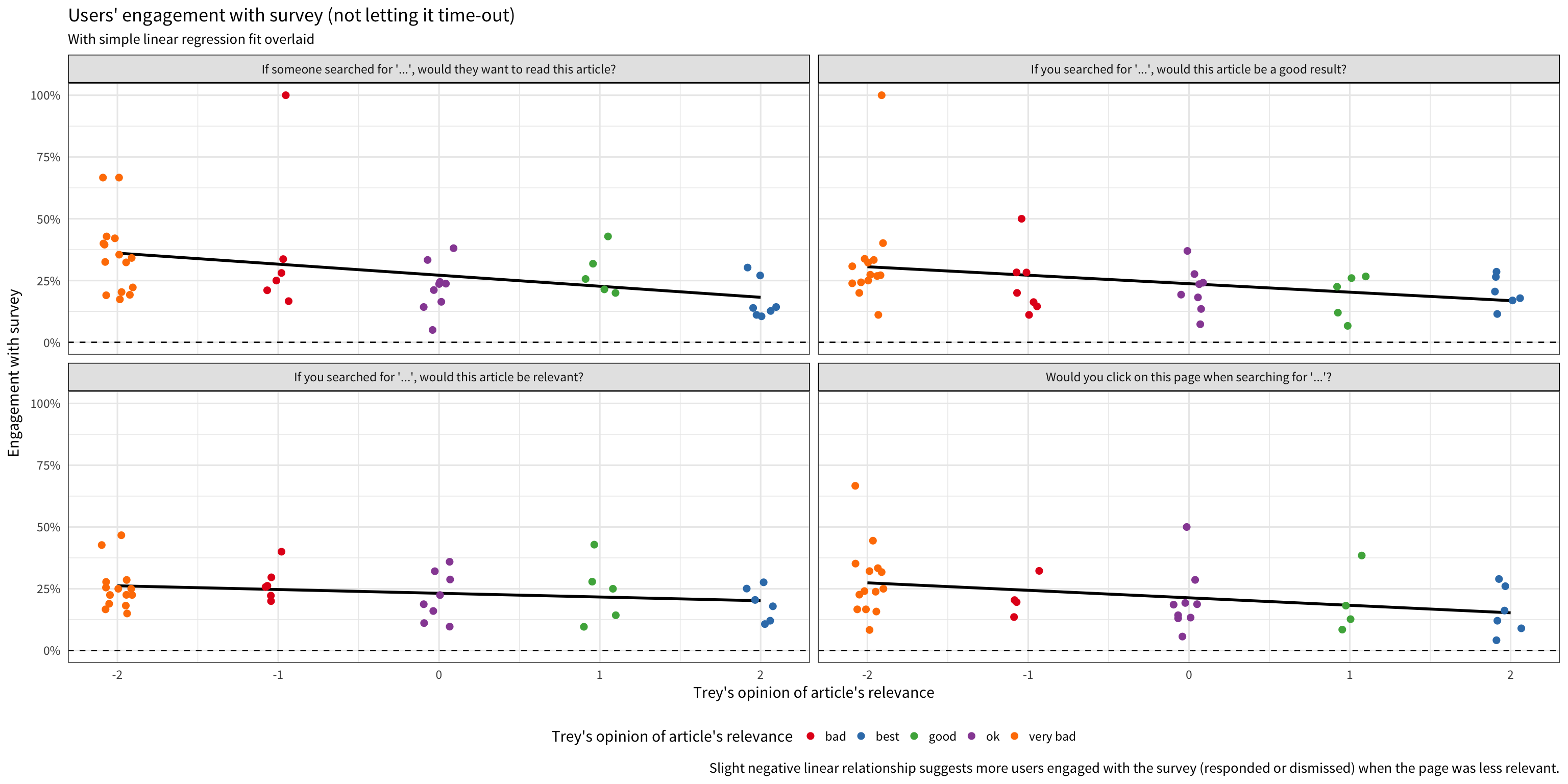↑ Top of section ##### Scoring Method A $\text{Score} = \frac{\#\{\text{response: yes}\} - \#\{\text{response: no}\}}{\#\{\text{response: yes/no}\} + 1}$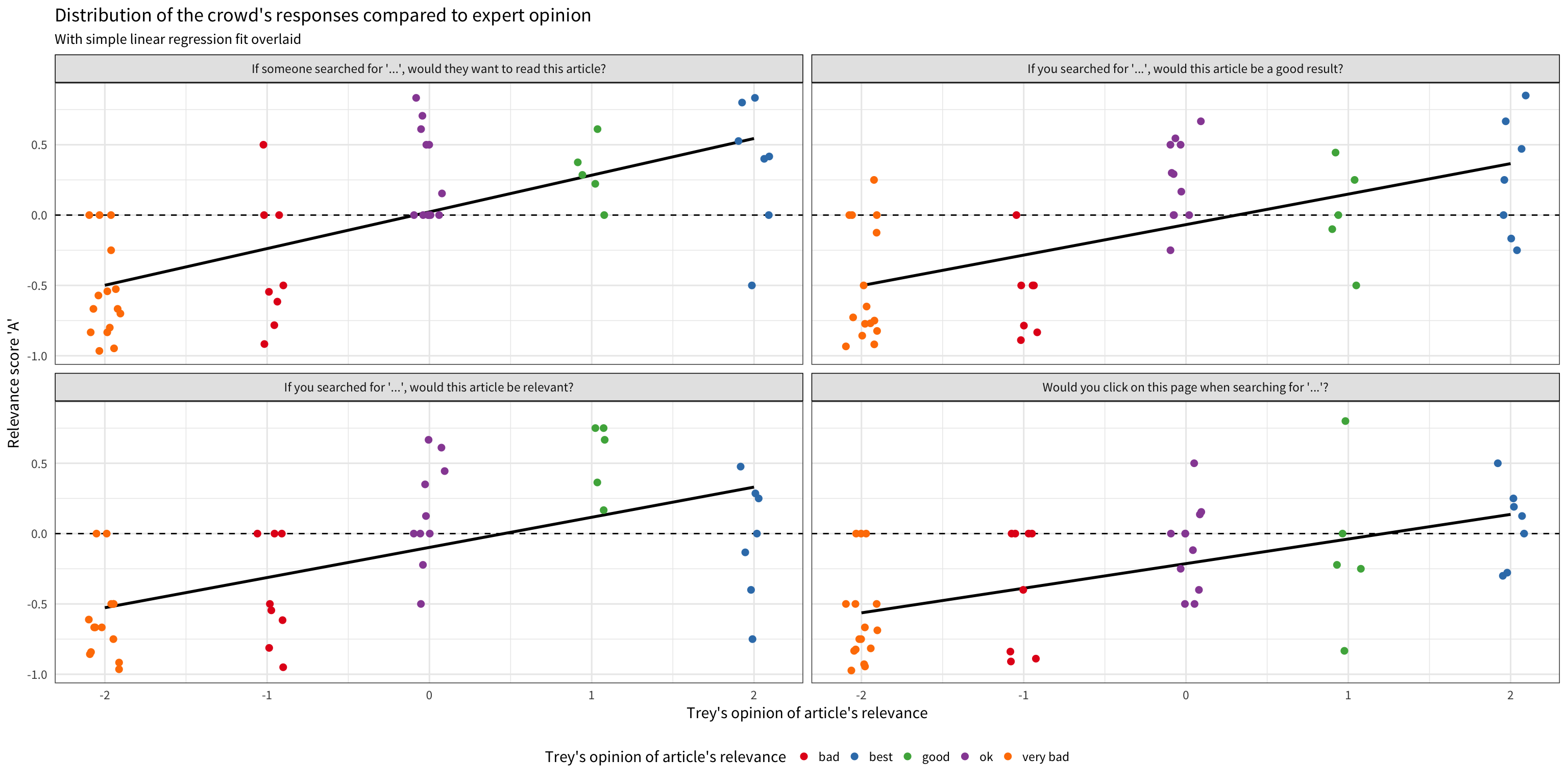##### Scoring Method B $\text{Score} = \frac{\#\{\text{response: yes}\} - \#\{\text{response: no}\} + \#\{\text{response: unsure}\}/2}{\#\{\text{response: yes/no/unsure}\} + 1}$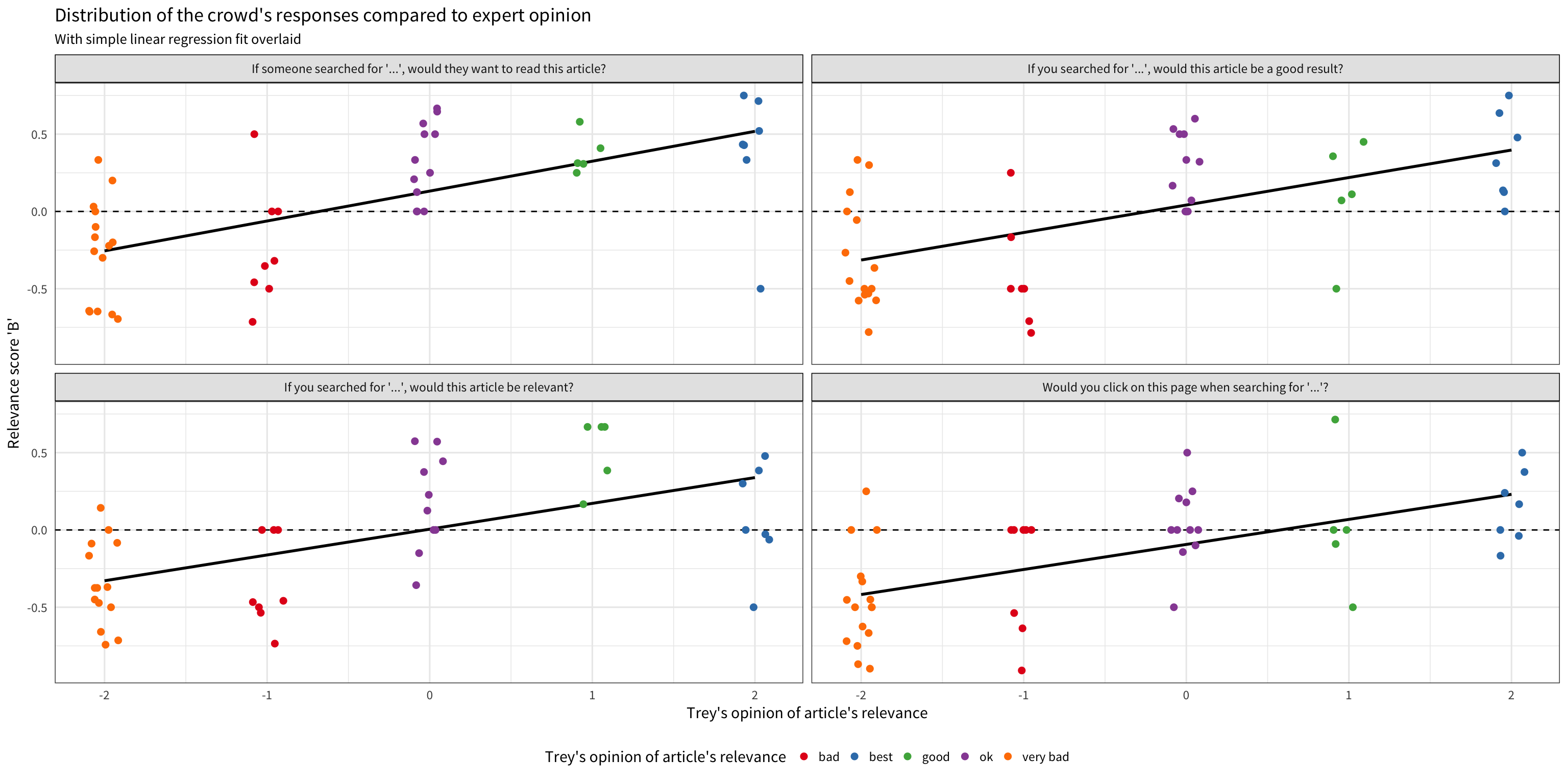↑ Top of section #### Survey Responses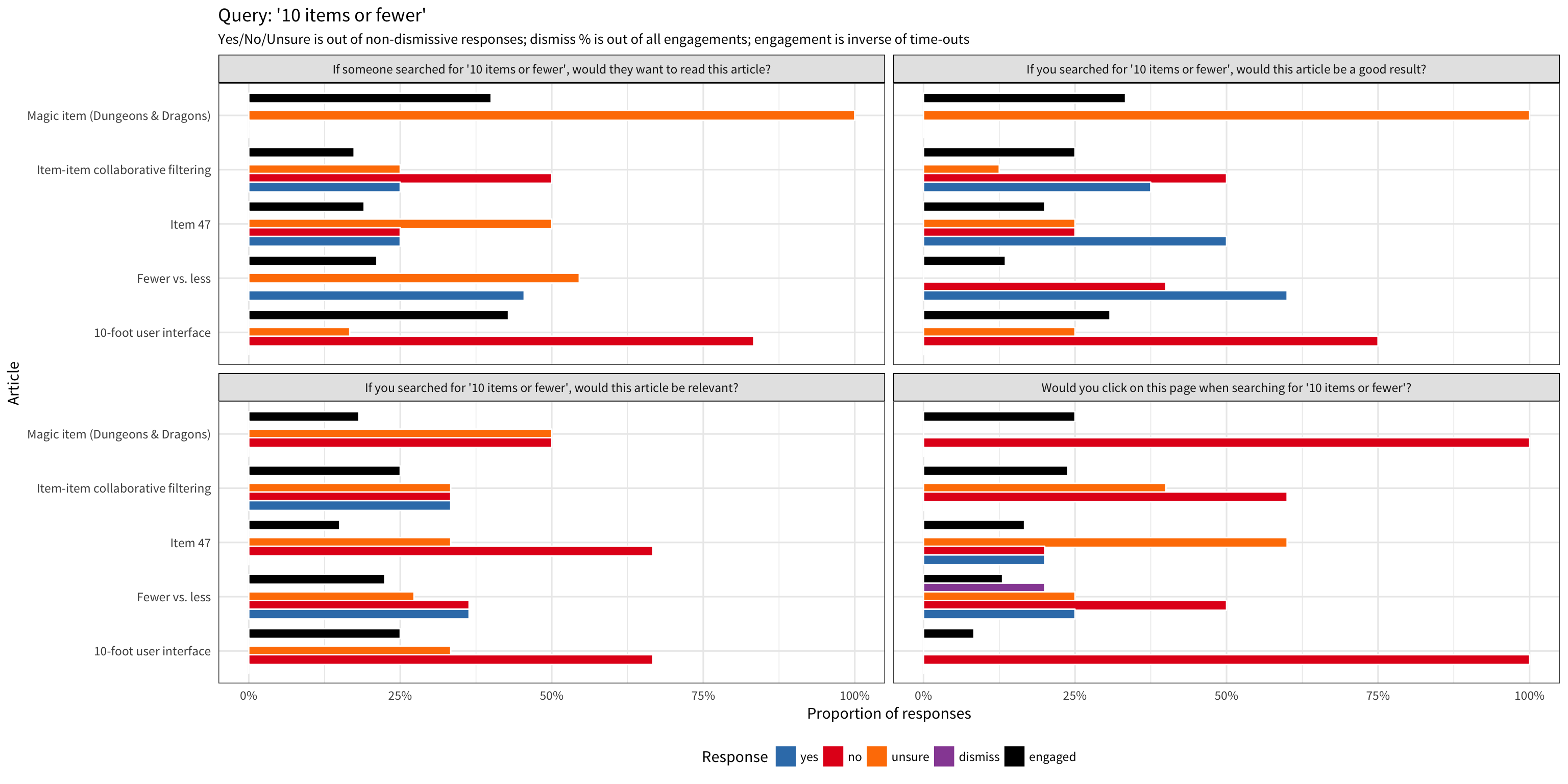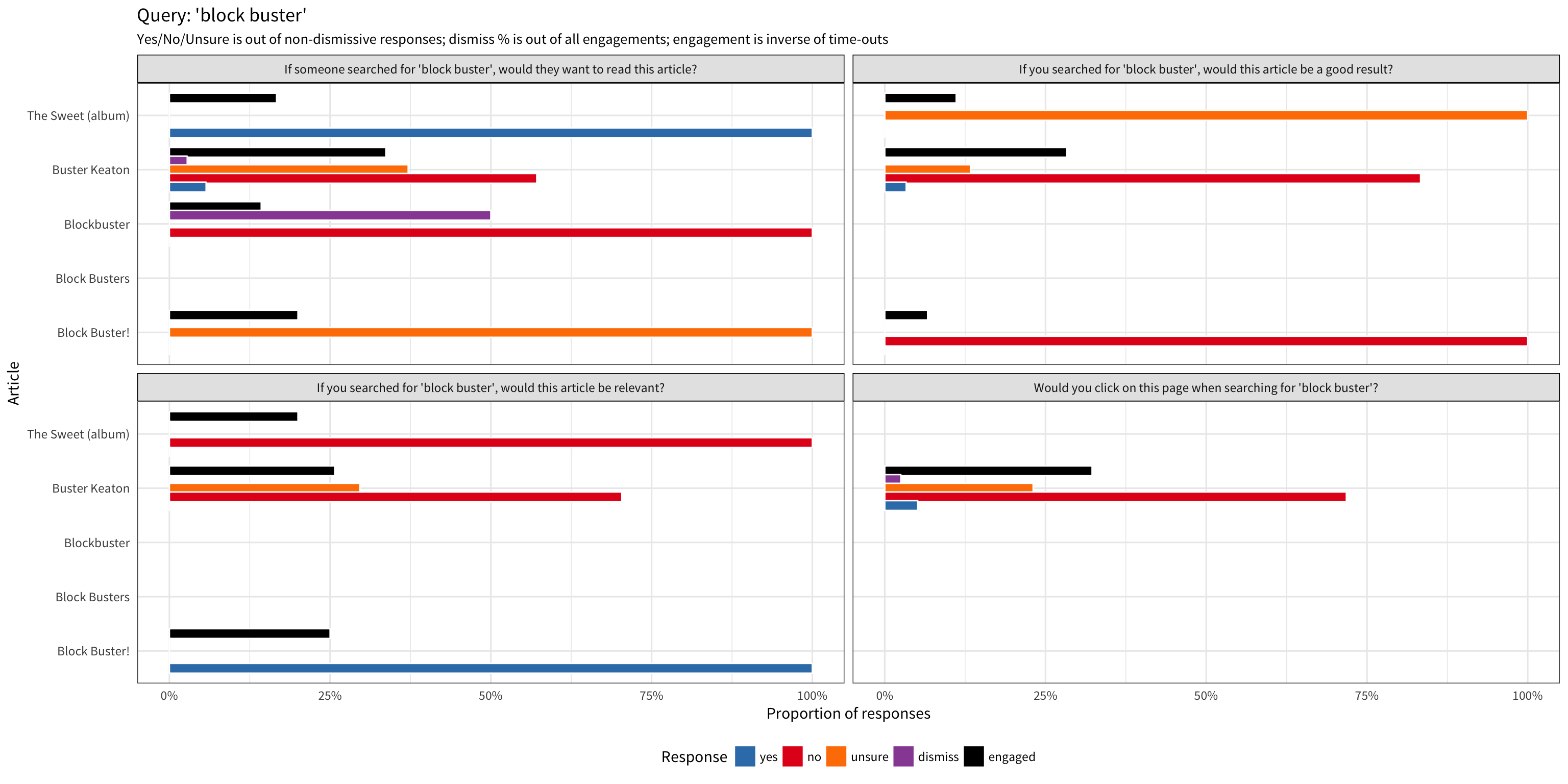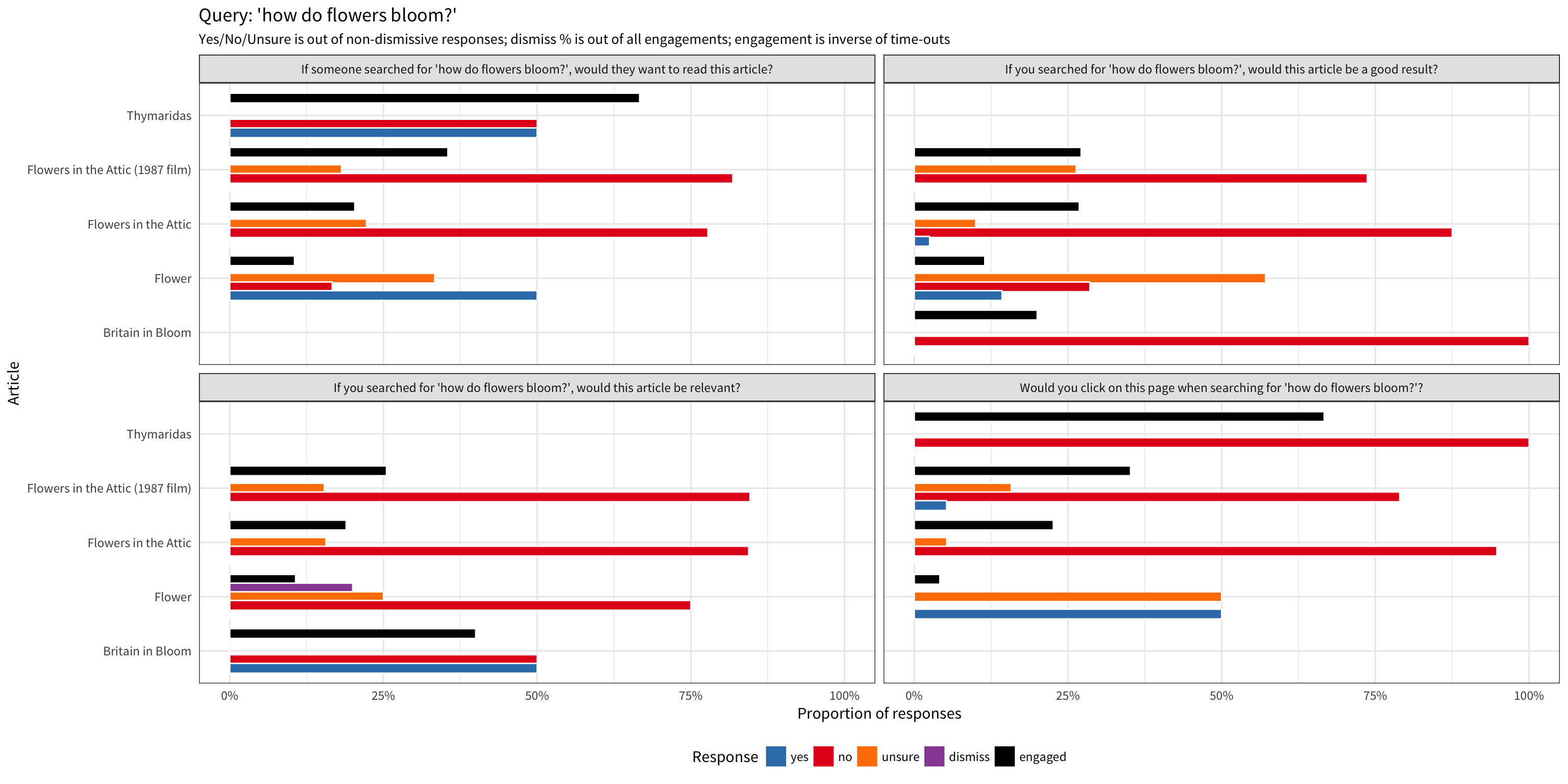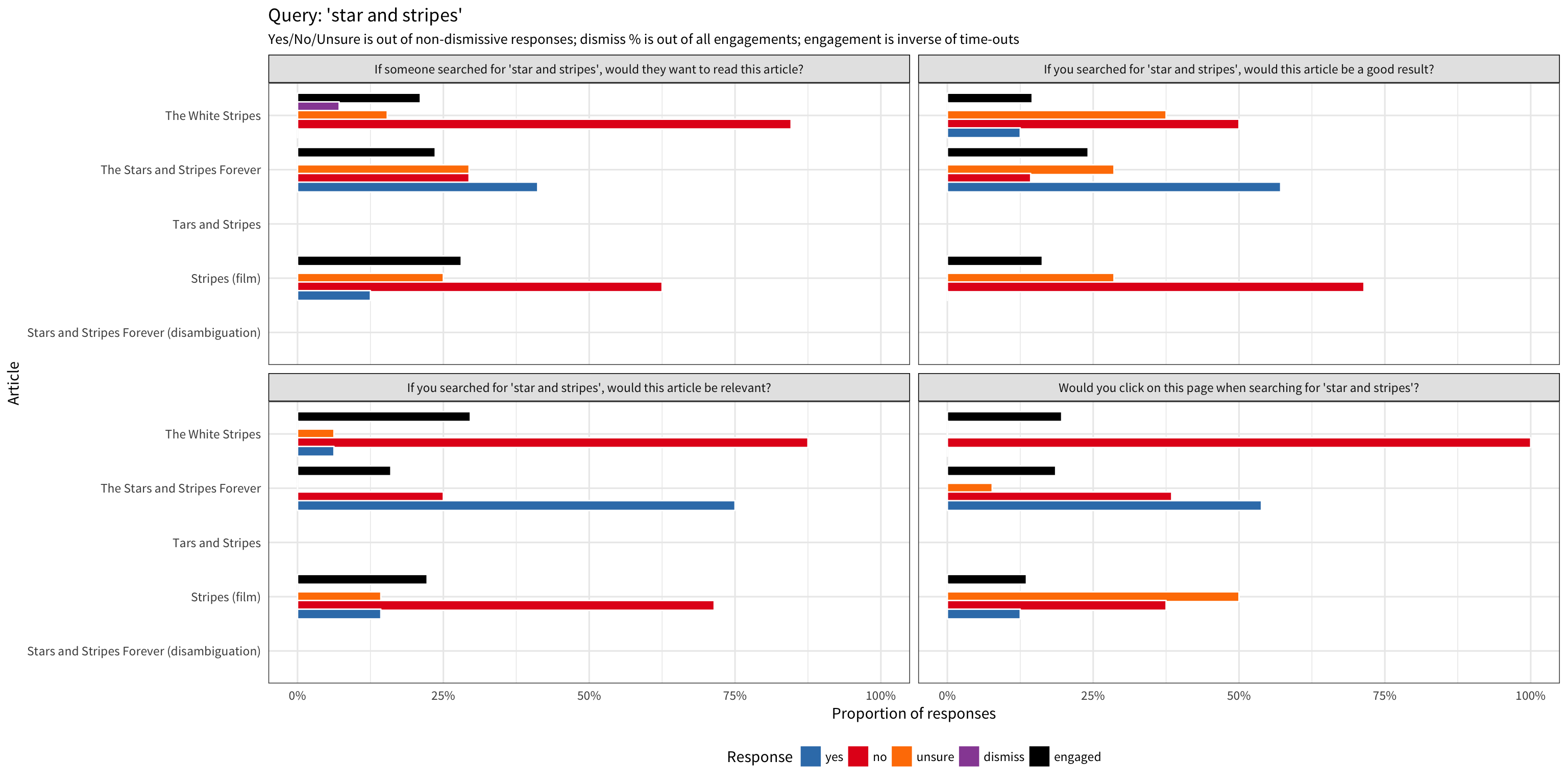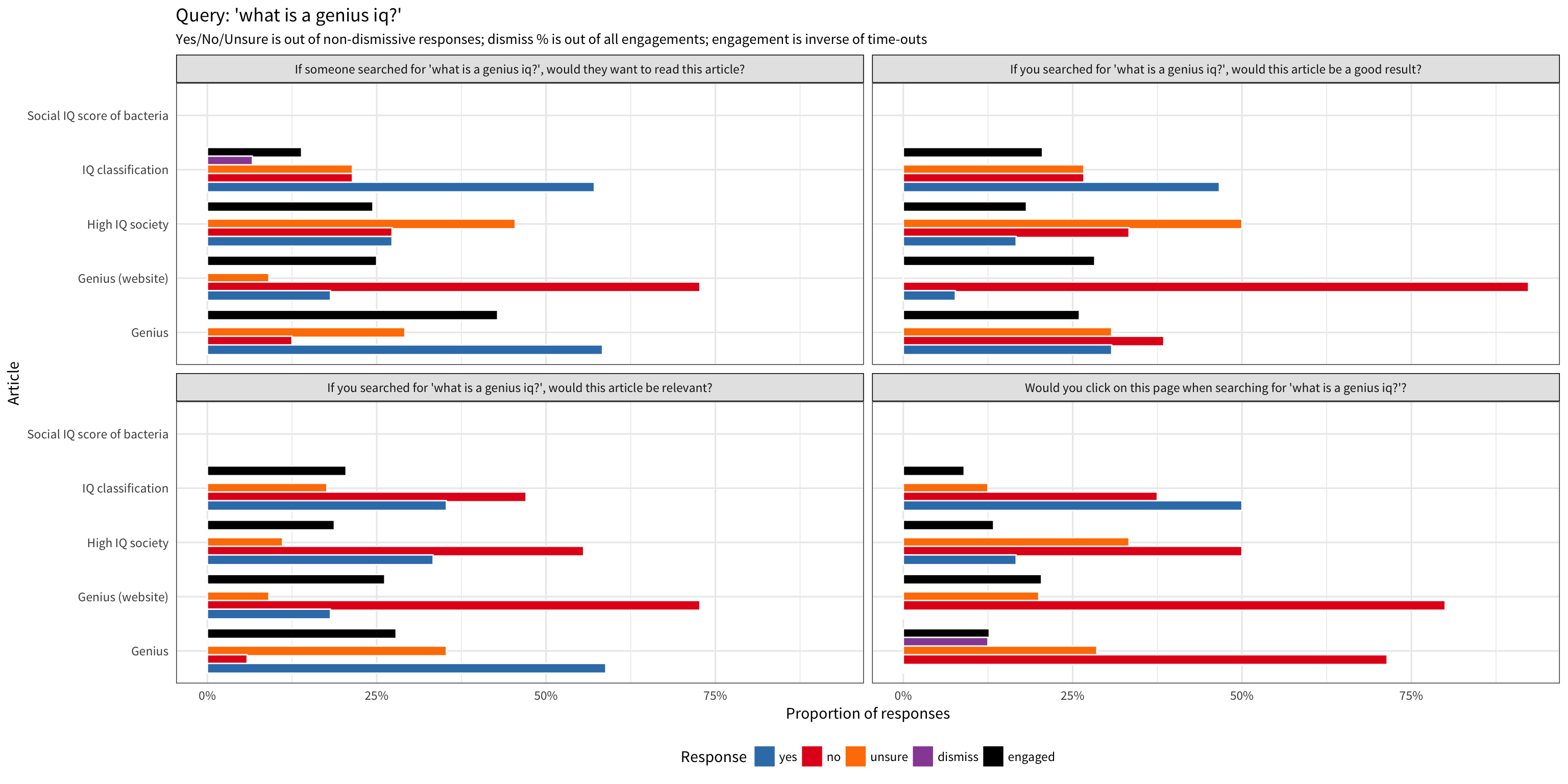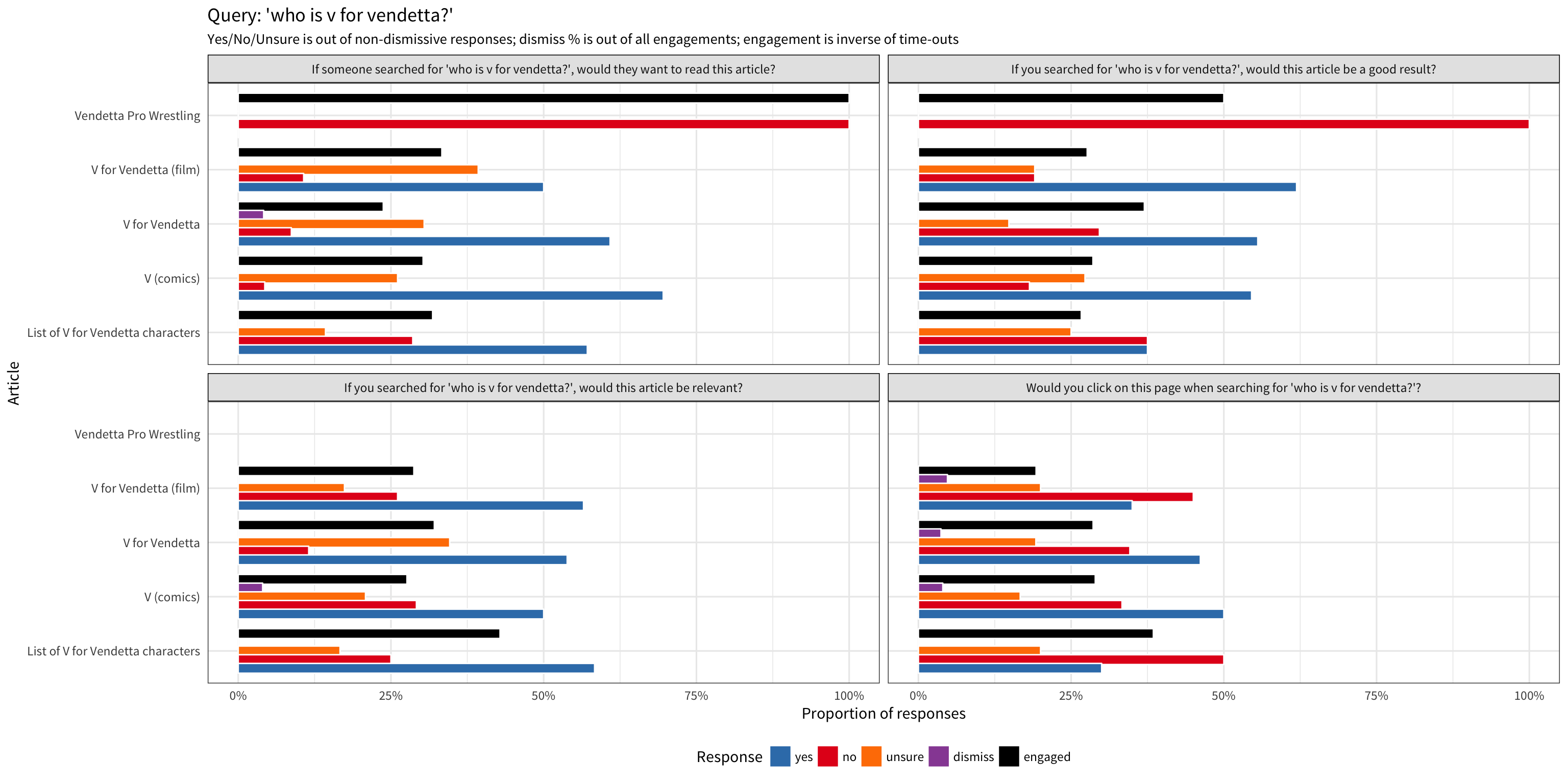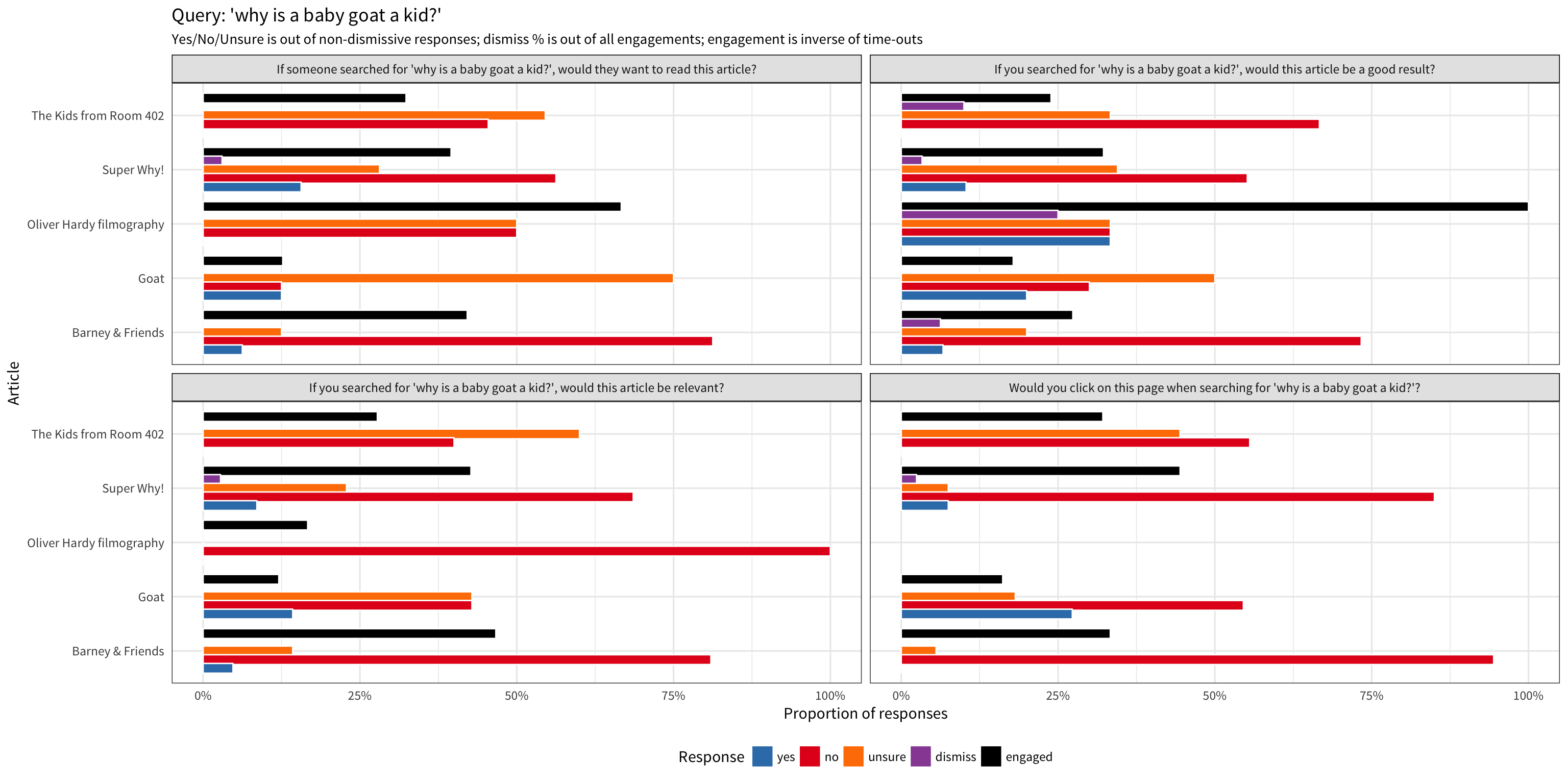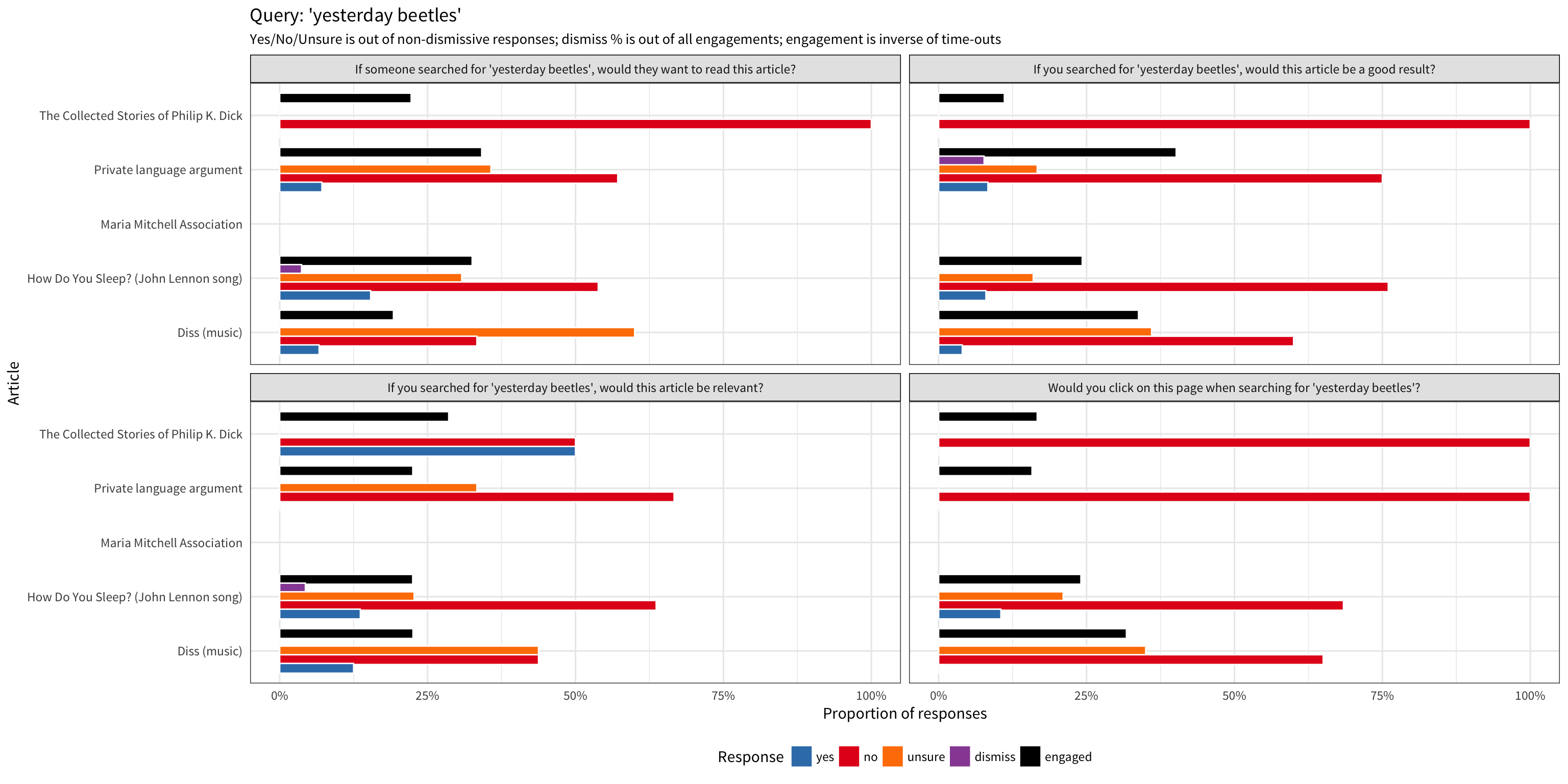↑ Top of section #### Relevance Predictions set.seed(42) ratings_second <- responses_second %>% dplyr::mutate( question = as.numeric(factor(question, levels = c( "Would you click on this page when searching for '...'?", "If you searched for '...', would this article be a good result?", "If you searched for '...', would this article be relevant?", "If someone searched for '...', would they want to read this article?" ))) ) %>% dplyr::group_by(query, article, choice, question) %>% dplyr::tally() %>% dplyr::ungroup() %>% tidyr::spread(choice, n, fill = 0) %>% dplyr::mutate( total = yes + no + unsure + 1, engaged = yes + no + unsure + dismiss, score_A = (yes - no) / (yes + no + 1), score_B = (yes - no + unsure / 2) / total, engagement = (engaged + dismiss) / (total + dismiss + timeout), # Normalized versions: score_A_norm = (score_A - mean(score_A)) / sd(score_A), score_B_norm = (score_B - mean(score_B)) / sd(score_B), engagement_norm = (engagement - mean(engagement)) / sd(engagement) ) %>% dplyr::left_join(trey, by = c("query", "article")) %>% dplyr::mutate( irrelevant = as.numeric(opinion %in% c("very bad", "bad")), ok_or_better = as.numeric(opinion %in% c("ok", "good", "best")), relevant = as.numeric(opinion %in% c("good", "best")), very_bad = as.numeric(opinion == "very bad"), bad = as.numeric(opinion == "bad"), ok = as.numeric(opinion == "ok"), good = as.numeric(opinion == "good"), best = as.numeric(opinion == "best"), opinion2 = factor(dplyr::case_when( opinion %in% c("very bad", "bad") ~ "bad", opinion %in% c("ok", "good", "best") ~ "ok or better" ), levels = c("bad", "ok or better")), opinion3 = factor(dplyr::case_when( opinion %in% c("very bad", "bad") ~ "bad", opinion %in% c("good", "best") ~ "good", opinion == "ok" ~ "ok" ), levels = c("bad", "ok", "good")), opinion5 = factor(opinion, levels = c("very bad", "bad", "ok", "good", "best")) ) %>% split(., .$question) %>%
lapply(function(df) {
training_idx <- sample.int(nrow(df), 0.7 * nrow(df), replace = FALSE)
testing_idx <- setdiff(1:nrow(df), training_idx)
return(list(train = df[training_idx, ], test = df[testing_idx, ]))
})
data_frame <- function(...) {
return(data.frame(..., stringsAsFactors = FALSE))
}
set.seed(0)
logistic_regression <- dplyr::bind_rows(list(
"2" = dplyr::bind_rows(lapply(ratings_second, function(question) {
lr_A <- glm(opinion2 ~ score_A + engagement, data = question$train, family = binomial()) predictions_A <- predict(lr_A, question$test[, c("score_A", "engagement")], type = "response")
lr_B <- glm(opinion2 ~ score_B + engagement, data = question$train, family = binomial()) predictions_B <- predict(lr_B, question$test[, c("score_B", "engagement")], type = "response")
accuracy <- list(
A = data_frame(accuracy = caret::confusionMatrix(
factor(predictions_A > 0.5, c(FALSE, TRUE), levels(question$test$opinion2)),
reference = question$test$opinion2
)$overall["Accuracy"]), B = data_frame(accuracy = caret::confusionMatrix( factor(predictions_B > 0.5, c(FALSE, TRUE), levels(question$test$opinion2)), reference = question$test$opinion2 )$overall["Accuracy"])
)
return(dplyr::bind_rows(accuracy, .id = "scoring"))
}), .id = "question"),
"3" = dplyr::bind_rows(lapply(ratings_second, function(question) {
lr_A <- nnet::multinom(
opinion3 ~ score_A + engagement,
data = question$train, trace = FALSE ) predictions_A <- predict(lr_A, question$test[, c("score_A", "engagement")])
lr_B <- nnet::multinom(
opinion3 ~ score_B + engagement,
data = question$train, trace = FALSE ) predictions_B <- predict(lr_B, question$test[, c("score_B", "engagement")])
accuracy <- list(
A = data_frame(accuracy = caret::confusionMatrix(
predictions_A, reference = question$test$opinion3
)$overall["Accuracy"]), B = data_frame(accuracy = caret::confusionMatrix( predictions_B, reference = question$test$opinion3 )$overall["Accuracy"])
)
return(dplyr::bind_rows(accuracy, .id = "scoring"))
}), .id = "question"),
"5" = dplyr::bind_rows(lapply(ratings_second, function(question) {
lr_A <- nnet::multinom(
opinion5 ~ score_A + engagement,
data = question$train, trace = FALSE ) predictions_A <- predict(lr_A, question$test[, c("score_A", "engagement")])
lr_B <- nnet::multinom(
opinion5 ~ score_B + engagement,
data = question$train, trace = FALSE ) predictions_B <- predict(lr_B, question$test[, c("score_B", "engagement")])
accuracy <- list(
A = data_frame(accuracy = caret::confusionMatrix(
predictions_A, reference = question$test$opinion5
)$overall["Accuracy"]), B = data_frame(accuracy = caret::confusionMatrix( predictions_B, reference = question$test$opinion5 )$overall["Accuracy"])
)
return(dplyr::bind_rows(accuracy, .id = "scoring"))
}), .id = "question")
), .id = "categories")

random_forest <- dplyr::bind_rows(list(
"2" = dplyr::bind_rows(lapply(ratings_second, function(question) {
rf_A <- randomForest::randomForest(
opinion2 ~ score_A + engagement,
data = question$train, ntree = 1000 ) predictions_A <- predict(rf_A, question$test[, c("score_A", "engagement")])
rf_B <- randomForest::randomForest(
opinion2 ~ score_B + engagement,
data = question$train, ntree = 1000 ) predictions_B <- predict(rf_B, question$test[, c("score_B", "engagement")])
accuracy <- list(
A = data_frame(accuracy = caret::confusionMatrix(
predictions_A, reference = question$test$opinion2
)$overall["Accuracy"]), B = data_frame(accuracy = caret::confusionMatrix( predictions_B, reference = question$test$opinion2 )$overall["Accuracy"])
)
return(dplyr::bind_rows(accuracy, .id = "scoring"))
}), .id = "question"),
"3" = dplyr::bind_rows(lapply(ratings_second, function(question) {
rf_A <- randomForest::randomForest(
opinion3 ~ score_A + engagement,
data = question$train, ntree = 1000 ) predictions_A <- predict(rf_A, question$test[, c("score_A", "engagement")])
rf_B <- randomForest::randomForest(
opinion3 ~ score_B + engagement,
data = question$train, ntree = 1000 ) predictions_B <- predict(rf_B, question$test[, c("score_B", "engagement")])
accuracy <- list(
A = data_frame(accuracy = caret::confusionMatrix(
predictions_A, reference = question$test$opinion3
)$overall["Accuracy"]), B = data_frame(accuracy = caret::confusionMatrix( predictions_B, reference = question$test$opinion3 )$overall["Accuracy"])
)
return(dplyr::bind_rows(accuracy, .id = "scoring"))
}), .id = "question"),
"5" = dplyr::bind_rows(lapply(ratings_second, function(question) {
rf_A <- randomForest::randomForest(
opinion5 ~ score_A + engagement,
data = question$train, ntree = 1000 ) predictions_A <- predict(rf_A, question$test[, c("score_A", "engagement")])
rf_B <- randomForest::randomForest(
opinion5 ~ score_B + engagement,
data = question$train, ntree = 1000 ) predictions_B <- predict(rf_B, question$test[, c("score_B", "engagement")])
accuracy <- list(
A = data_frame(accuracy = caret::confusionMatrix(
predictions_A, reference = question$test$opinion5
)$overall["Accuracy"]), B = data_frame(accuracy = caret::confusionMatrix( predictions_B, reference = question$test$opinion5 )$overall["Accuracy"])
)
return(dplyr::bind_rows(accuracy, .id = "scoring"))
}), .id = "question")
), .id = "categories")

neural_net <- dplyr::bind_rows(list(
"2" = dplyr::bind_rows(lapply(ratings_second, function(question) {
nn_A <- neuralnet::neuralnet(
irrelevant + ok_or_better ~ score_A + engagement,
data = question$train, hidden = c(5, 3), stepmax = 1e6 ) predictions_A <- factor( c("bad", "ok or better")[apply(neuralnet::compute(nn_A, question$test[, c("score_A", "engagement")])$net.result, 1, which.max)], levels = c("bad", "ok or better") ) nn_B <- neuralnet::neuralnet( irrelevant + ok_or_better ~ score_B + engagement, data = question$train,
hidden = c(5, 3), stepmax = 1e6
)
predictions_B <- factor(
c("bad", "ok or better")[apply(neuralnet::compute(nn_B, question$test[, c("score_B", "engagement")])$net.result, 1, which.max)],
levels = c("bad", "ok or better")
)
accuracy <- list(
A = data_frame(accuracy = caret::confusionMatrix(
predictions_A, reference = question$test$opinion2
)$overall["Accuracy"]), B = data_frame(accuracy = caret::confusionMatrix( predictions_B, reference = question$test$opinion2 )$overall["Accuracy"])
)
return(dplyr::bind_rows(accuracy, .id = "scoring"))
}), .id = "question"),
"3" = dplyr::bind_rows(lapply(ratings_second, function(question) {
nn_A <- neuralnet::neuralnet(
irrelevant + ok + relevant ~ score_A + engagement,
data = question$train, hidden = c(5, 3), stepmax = 1e6 ) predictions_A <- factor( c("bad", "ok", "good")[apply(neuralnet::compute(nn_A, question$test[, c("score_A", "engagement")])$net.result, 1, which.max)], levels = c("bad", "ok", "good") ) nn_B <- neuralnet::neuralnet( irrelevant + ok + relevant ~ score_B + engagement, data = question$train,
hidden = c(5, 3), stepmax = 1e6
)
predictions_B <- factor(
c("bad", "ok", "good")[apply(neuralnet::compute(nn_B, question$test[, c("score_B", "engagement")])$net.result, 1, which.max)],
)
accuracy <- list(
A = data_frame(accuracy = caret::confusionMatrix(
predictions_A, reference = question$test$opinion3
)$overall["Accuracy"]), B = data_frame(accuracy = caret::confusionMatrix( predictions_B, reference = question$test$opinion3 )$overall["Accuracy"])
)
return(dplyr::bind_rows(accuracy, .id = "scoring"))
}), .id = "question"),
"5" = dplyr::bind_rows(lapply(ratings_second, function(question) {
nn_A <- neuralnet::neuralnet(
very_bad + bad + ok + good + best ~ score_A + engagement,
data = question$train, hidden = c(5, 3), stepmax = 1e6 ) predictions_A <- factor( c("very bad", "bad", "ok", "good", "best")[apply(neuralnet::compute(nn_A, question$test[, c("score_A", "engagement")])$net.result, 1, which.max)], levels = c("very bad", "bad", "ok", "good", "best") ) nn_B <- neuralnet::neuralnet( very_bad + bad + ok + good + best ~ score_B + engagement, data = question$train,
hidden = c(5, 3), stepmax = 1e6
)
predictions_B <- factor(
c("very bad", "bad", "ok", "good", "best")[apply(neuralnet::compute(nn_B, question$test[, c("score_A", "engagement")])$net.result, 1, which.max)],
)
accuracy <- list(
A = data_frame(accuracy = caret::confusionMatrix(
predictions_A, reference = question$test$opinion5
)$overall["Accuracy"]), B = data_frame(accuracy = caret::confusionMatrix( predictions_B, reference = question$test$opinion5 )$overall["Accuracy"])
)
return(dplyr::bind_rows(accuracy, .id = "scoring"))
}), .id = "question")
), .id = "categories")

naive_bayes <- dplyr::bind_rows(list(
"2" = dplyr::bind_rows(lapply(ratings_second, function(question) {
nb_A <- e1071::naiveBayes(
opinion2 ~ score_A + engagement,
data = question$train ) predictions_A <- predict(nb_A, question$test[, c("score_A", "engagement")])
nb_B <- e1071::naiveBayes(
opinion2 ~ score_B + engagement,
data = question$train ) predictions_B <- predict(nb_B, question$test[, c("score_A", "engagement")])
accuracy <- list(
A = data_frame(accuracy = caret::confusionMatrix(
predictions_A, reference = question$test$opinion2
)$overall["Accuracy"]), B = data_frame(accuracy = caret::confusionMatrix( predictions_B, reference = question$test$opinion2 )$overall["Accuracy"])
)
return(dplyr::bind_rows(accuracy, .id = "scoring"))
}), .id = "question"),
"3" = dplyr::bind_rows(lapply(ratings_second, function(question) {
nb_A <- e1071::naiveBayes(
opinion3 ~ score_A + engagement,
data = question$train ) predictions_A <- predict(nb_A, question$test[, c("score_A", "engagement")])
nb_B <- e1071::naiveBayes(
opinion3 ~ score_B + engagement,
data = question$train ) predictions_B <- predict(nb_B, question$test[, c("score_A", "engagement")])
accuracy <- list(
A = data_frame(accuracy = caret::confusionMatrix(
predictions_A, reference = question$test$opinion3
)$overall["Accuracy"]), B = data_frame(accuracy = caret::confusionMatrix( predictions_B, reference = question$test$opinion3 )$overall["Accuracy"])
)
return(dplyr::bind_rows(accuracy, .id = "scoring"))
}), .id = "question"),
"5" = dplyr::bind_rows(lapply(ratings_second, function(question) {
nb_A <- e1071::naiveBayes(
opinion5 ~ score_A + engagement,
data = question$train ) predictions_A <- predict(nb_A, question$test[, c("score_A", "engagement")])
nb_B <- e1071::naiveBayes(
opinion5 ~ score_B + engagement,
data = question$train ) predictions_B <- predict(nb_B, question$test[, c("score_A", "engagement")])
accuracy <- list(
A = data_frame(accuracy = caret::confusionMatrix(
predictions_A, reference = question$test$opinion5
)$overall["Accuracy"]), B = data_frame(accuracy = caret::confusionMatrix( predictions_B, reference = question$test$opinion5 )$overall["Accuracy"])
)
return(dplyr::bind_rows(accuracy, .id = "scoring"))
}), .id = "question")
), .id = "categories")

"2" = dplyr::bind_rows(lapply(ratings_second, function(question) {
xgb_A <- xgboost::xgboost(
data = as.matrix(question$train[, c("score_A", "engagement")]), label = as.numeric(question$train$opinion2) - 1, objective = "binary:logistic", nrounds = 100, nthread = 4, verbose = 0 ) predictions_A <- predict(xgb_A, as.matrix(question$test[, c("score_A", "engagement")]))
xgb_B <- xgboost::xgboost(
data = as.matrix(question$train[, c("score_B", "engagement")]), label = as.numeric(question$train$opinion2) - 1, objective = "binary:logistic", nrounds = 100, nthread = 4, verbose = 0 ) predictions_B <- predict(xgb_B, as.matrix(question$test[, c("score_B", "engagement")]))
accuracy <- list(
A = data_frame(accuracy = caret::confusionMatrix(
factor(predictions_A > 0.5, c(FALSE, TRUE), c("bad", "ok or better")),
reference = question$test$opinion2
)$overall["Accuracy"]), B = data_frame(accuracy = caret::confusionMatrix( factor(predictions_B > 0.5, c(FALSE, TRUE), c("bad", "ok or better")), reference = question$test$opinion2 )$overall["Accuracy"])
)
return(dplyr::bind_rows(accuracy, .id = "scoring"))
}), .id = "question"),
"3" = dplyr::bind_rows(lapply(ratings_second, function(question) {
xgb_A <- xgboost::xgboost(
data = as.matrix(question$train[, c("score_A", "engagement")]), label = as.numeric(question$train$opinion3) - 1, num_class = 3, objective = "multi:softmax", nrounds = 100, nthread = 4, verbose = 0 ) predictions_A <- predict(xgb_A, as.matrix(question$test[, c("score_A", "engagement")]))
xgb_B <- xgboost::xgboost(
data = as.matrix(question$train[, c("score_B", "engagement")]), label = as.numeric(question$train$opinion3) - 1, num_class = 3, objective = "multi:softmax", nrounds = 100, nthread = 4, verbose = 0 ) predictions_B <- predict(xgb_B, as.matrix(question$test[, c("score_B", "engagement")]))
accuracy <- list(
A = data_frame(accuracy = caret::confusionMatrix(
factor(predictions_A, 0:2, levels(question$test$opinion3)),
reference = question$test$opinion3
)$overall["Accuracy"]), B = data_frame(accuracy = caret::confusionMatrix( factor(predictions_B, 0:2, levels(question$test$opinion3)), reference = question$test$opinion3 )$overall["Accuracy"])
)
return(dplyr::bind_rows(accuracy, .id = "scoring"))
}), .id = "question"),
"5" = dplyr::bind_rows(lapply(ratings_second, function(question) {
xgb_A <- xgboost::xgboost(
data = as.matrix(question$train[, c("score_A", "engagement")]), label = as.numeric(question$train$opinion5) - 1, num_class = 5, objective = "multi:softmax", nrounds = 100, nthread = 4, verbose = 0 ) predictions_A <- predict(xgb_A, as.matrix(question$test[, c("score_A", "engagement")]))
xgb_B <- xgboost::xgboost(
data = as.matrix(question$train[, c("score_B", "engagement")]), label = as.numeric(question$train$opinion5) - 1, num_class = 5, objective = "multi:softmax", nrounds = 100, nthread = 4, verbose = 0 ) predictions_B <- predict(xgb_B, as.matrix(question$test[, c("score_B", "engagement")]))
accuracy <- list(
A = data_frame(accuracy = caret::confusionMatrix(
factor(predictions_A, 0:4, levels(question$test$opinion5)),
reference = question$test$opinion5
)$overall["Accuracy"]), B = data_frame(accuracy = caret::confusionMatrix( factor(predictions_B, 0:4, levels(question$test$opinion5)), reference = question$test$opinion5 )$overall["Accuracy"])
)
return(dplyr::bind_rows(accuracy, .id = "scoring"))
}), .id = "question")
), .id = "categories")

↑ Top of section

## Conclusion

Using relatively few article judgements, we are able to train models that perform remarkably well given the amount of data. That is, using a larger set of articles for which we have an “expert opinion” regarding their relevance to a certain search query, we will be able to train a model that accurately predicts an article’s relevance just from users’ survey responses and engagement with the survey. This will enable us to use aggregated public opinion to rank a large volume of articles.

Using binary classification (irrelevant/relevant), we can ask the algorithms to return predicted probabilities instead of predicted classes. Assuming the model has exceptional accuracy, we can then feed queries and rankings (predicted from users’ survey responses) as training data into our learning-to-rank (LTR) endeavor.1

In a presentation of this work,2 Dario Taraborelli (Head of Wikimedia Research) brought up the valid point of how the question wording (“relevant to you” vs. “relevant to people”) primes the respondent differently and could yield different results. It was interesting, then, to see question 4 (“If someone searched for ‘…’, would they want to read this article?”) show up in both tests as the question whose responses yielded the better classification performances.

## Discussion

As this was a proof of concept / MVP, we did not apply a lot of rigor to certain aspects. For example, we did not have multiple experts agree on the articles’ relevance, but rather one person’s opinions were used as the gold standard. On the analysis side, a lot of the time was spent getting multiple classification algorithms running in different configuration combinations; so we were not able to include a more rigorous accuracy estimation approach like cross-validation. At the time of this report’s publication we are planning on launching a third test (T174106).

Then there’s the issue of what this looks like in production. For deploying this on Wikipedia, we might want to specifically optimize for encyclopedic searches. For example, “barack obama birthdate” and “iphone 7 release date” are encyclopedic search queries, but “how do i 3d print a miniature santa claus?” is not. Unless we utilize natural language processing and machine learning to detect such queries, we cannot automate query selection by picking the most popular queries to ask users about. We would also need to decide whether to train relevance models on a per-wiki/project/language basis. That is, we cannot assume that the relationship between survey responses, engagement, and relevance is the same on German Wikipedia, French Wikisource, and Wikidata.

Filtering for encyclopedicity is just one thing that human reviewers will have to do. We will have to curate the queries anyway because we need to filter for personally identifiable information (PII), gibberish, and queries in the wrong language. However, although manually reviewing 500 queries takes a few hours, we can easily leverage that into 25K-50K crowd-sourced relevance judgements (50-100 results per query) in a relatively short time, whereas the more tedious Discernatron has only gotten ~7500 (150 queries * ~50 results per query) over the course of about a year.